• 这一篇需要长时间Update，大致框架的想法是根据不同图的类型分类，实例展示 – 归纳/语句 – Doc说明（功能和参数）。 Bar Plot Figure 1只需要简单的四组数据，实现： X轴label Bar颜色选择和数据标记 Title 去...


文章目录
Bar PlotScatter Matrix Plot3D Scatter PlotDecision Boundary PlotScatter Plot(I) K-NN classfication accuracy versus sensitivity to K(II) K-NN classfication accuracy versus sensitivity to train-test proportion

Bar Plot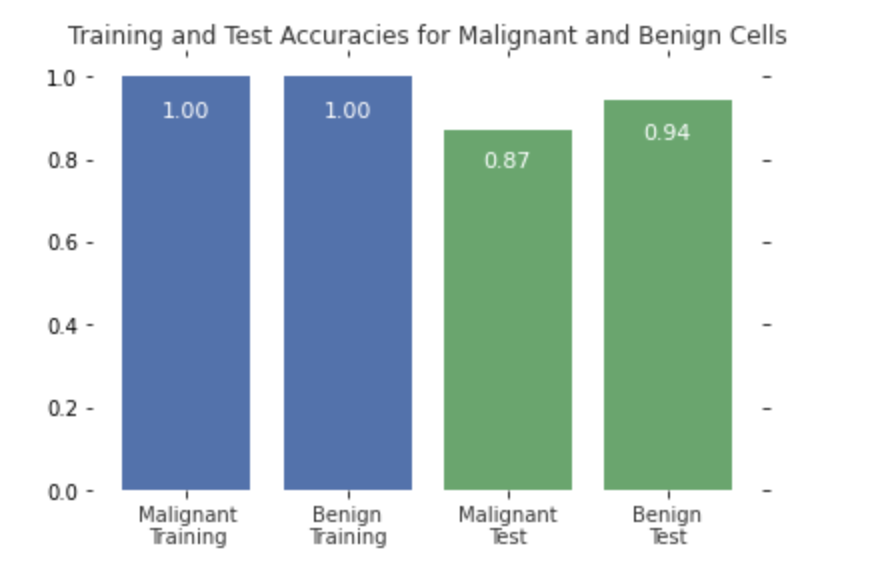Figure 1只需要简单的四组数据，实现：
X轴labelBar颜色选择和数据标记Title去框线
import matplotlib.pyplot as plt
plt.figure()
bars=plt.bar(np.arange(4),scores,color=['#4c72b0','#4c72b0','#55a868','#55a868'])

#label score onto the bar
for bar in bars:
height=bar.get_height()
plt.gca().text(bar.get_x()+ bar.get_width()/2,height*.90,'{0:.{1}f}'.format(height, 2),ha='center', color='w', fontsize=11)

#remove all the ticks (both axes), and tick labels on the Y axis
plt.tick_params(top='off', bottom='off', left='off', right='off', labelleft='off', labelbottom='on')

#remove the frame of the chart
for spine in plt.gca().spines.values():
spine.set_visible(False)

plt.xticks([0,1,2,3], ['Malignant\nTraining', 'Benign\nTraining', 'Malignant\nTest', 'Benign\nTest'], alpha=0.8);
plt.title('Training and Test Accuracies for Malignant and Benign Cells', alpha=0.8)

plt.figure() plt.bar() plt.gca().text() plt.gca().spines.values() spine.set_visible() plt.xticks() plt.title()
bar.get_height() bar.get_x() bar.get_width()
Scatter Matrix PlotData consists of 4 features + one label
# plotting a scatter matrix
from matplotlib import cm #color map

X = fruits[['height', 'width', 'mass', 'color_score']]
y = fruits['fruit_label']
X_train, X_test, y_train, y_test = train_test_split(X, y, random_state=0)

cmap = cm.get_cmap('gnuplot')
scatter = pd.scatter_matrix(X_train, c= y_train, marker = 'o', s=40, hist_kwds={'bins':15}, figsize=(7,7), cmap=cmap)

cm.get_cmap() pd.scatter_matrix()
3D Scatter Plot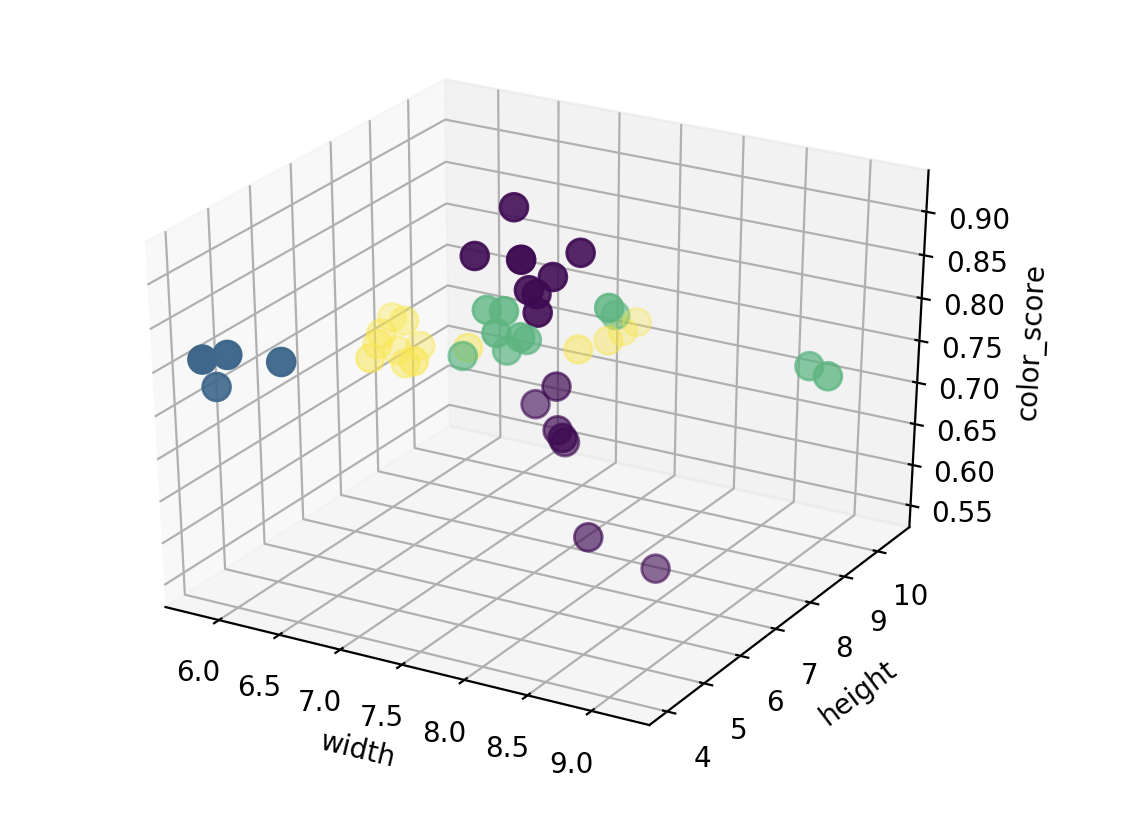data=features of 3+one label
# plotting a 3D scatter plot
from mpl_toolkits.mplot3d import Axes3D

fig = plt.figure()
ax = fig.add_subplot(111, projection = '3d')
ax.scatter(X_train['width'], X_train['height'], X_train['color_score'], c = y_train, marker = 'o', s=100)
ax.set_xlabel('width')
ax.set_ylabel('height')
ax.set_zlabel('color_score')
plt.show()

fig=plt.figure()
plt.show()
Decision Boundary Plot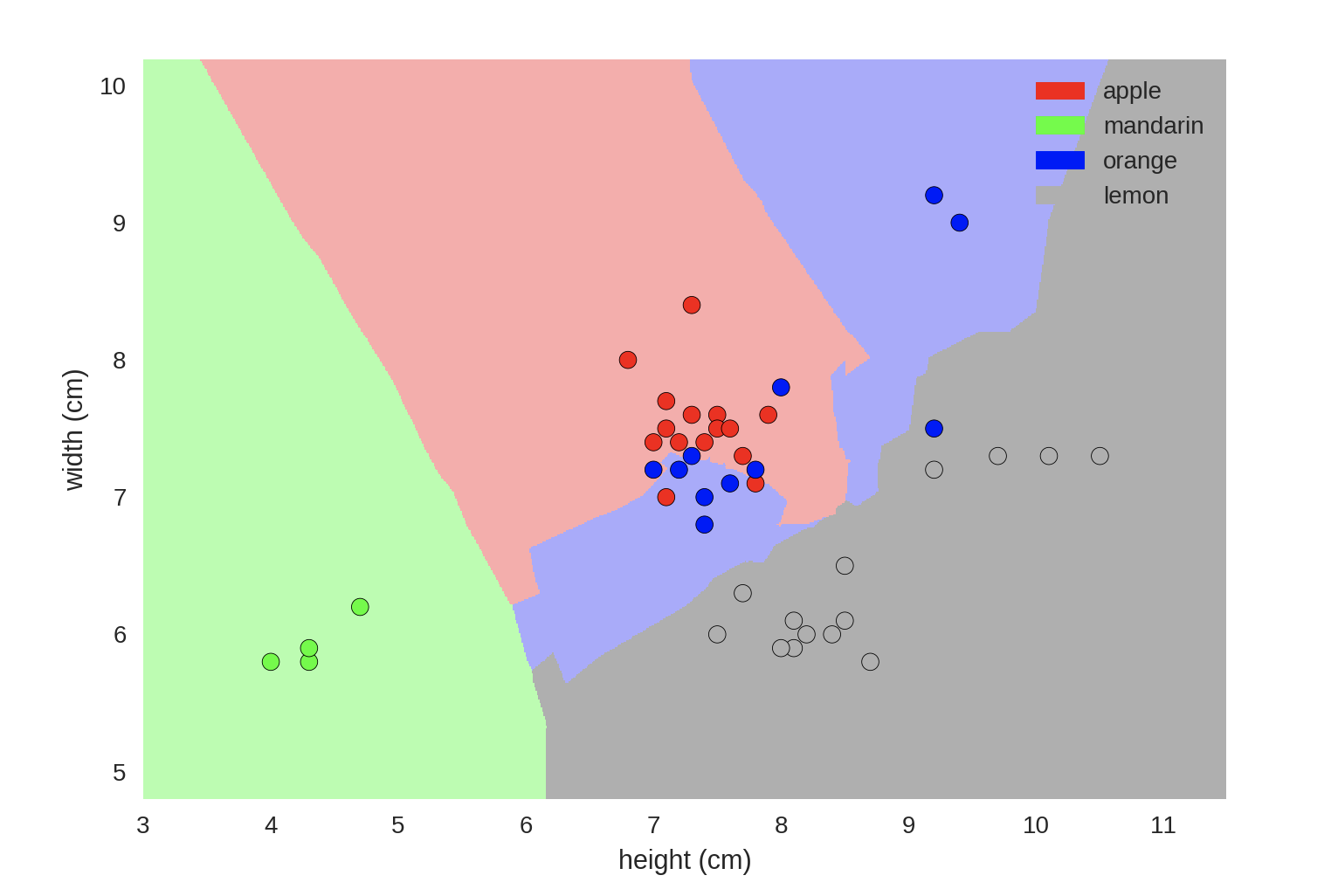没展示具体的实现代码
from adspy_shared_utilities import plot_fruit_knn

plot_fruit_knn(X_train, y_train, 5, 'uniform')   # we choose 5 nearest neighbors

Scatter Plot
(I) K-NN classfication accuracy versus sensitivity to K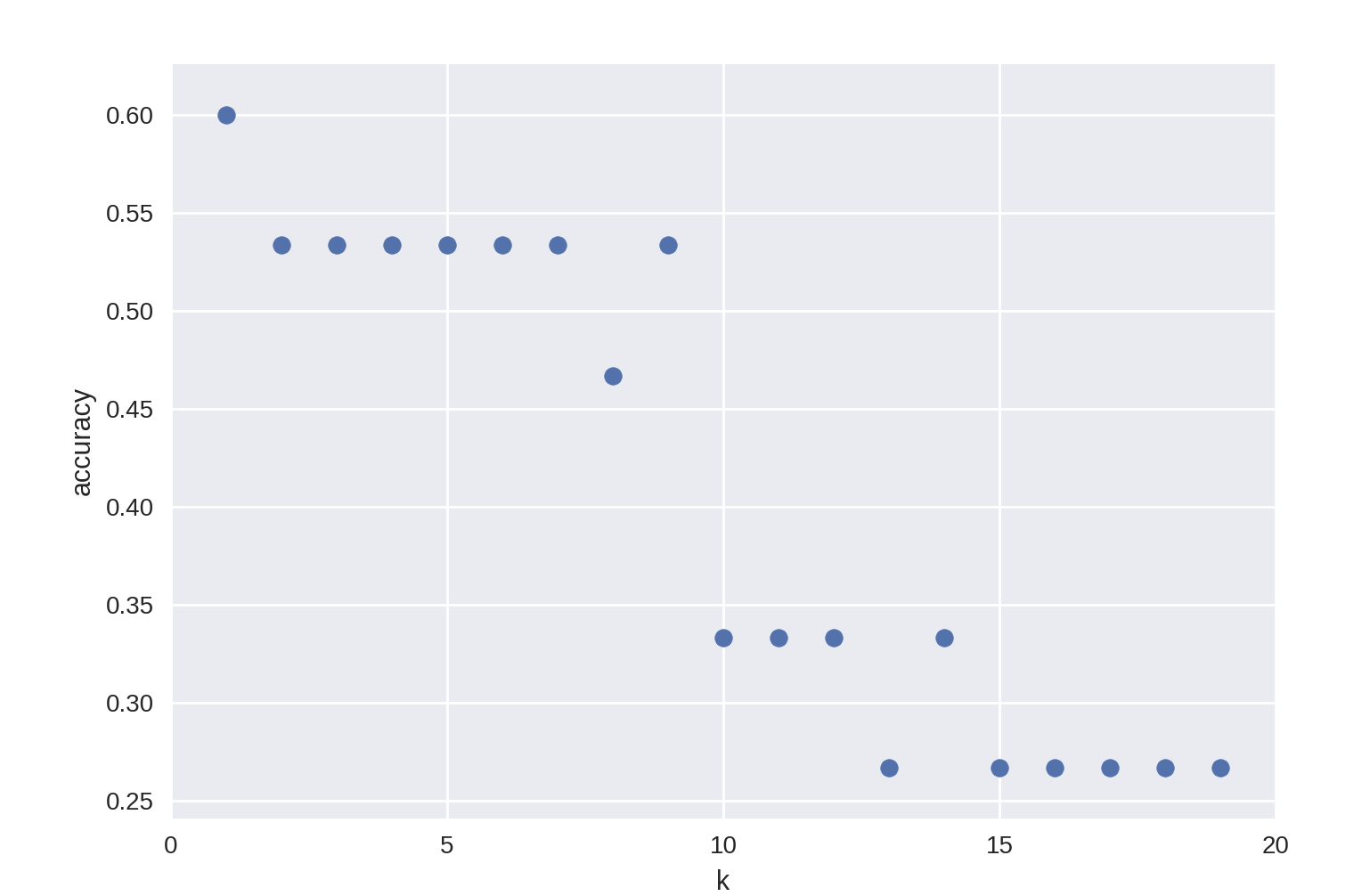20 pairs of data to choose K
import matplotlib.pyplot as plt
k_range = range(1,20)
scores = []

for k in k_range:
knn = KNeighborsClassifier(n_neighbors = k)
knn.fit(X_train, y_train)
scores.append(knn.score(X_test, y_test))

plt.figure()
plt.xlabel('k')
plt.ylabel('accuracy')
plt.scatter(k_range, scores)
plt.xticks([0,5,10,15,20]);

plt.figure() plt.scatter() plt.xlabel() plt.ylabel() plt.xticks()
(II) K-NN classfication accuracy versus sensitivity to train-test proportion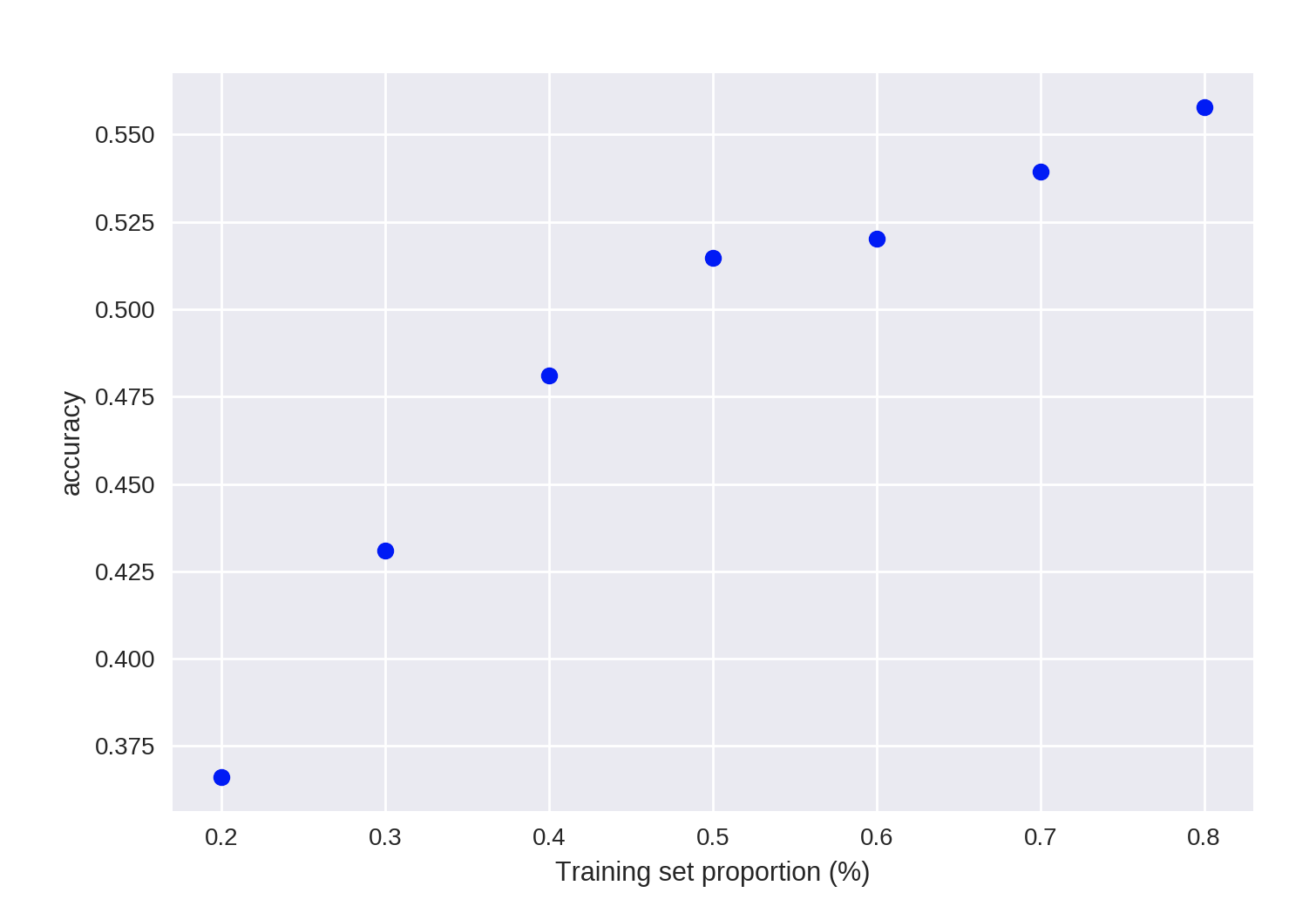7 pairs of data
import matplotlib.pyplot as plt
t = [0.8, 0.7, 0.6, 0.5, 0.4, 0.3, 0.2]

knn = KNeighborsClassifier(n_neighbors = 5)

plt.figure()

for s in t:

scores = []
for i in range(1,1000):
X_train, X_test, y_train, y_test = train_test_split(X, y, test_size = 1-s)
knn.fit(X_train, y_train)
scores.append(knn.score(X_test, y_test))
plt.plot(s, np.mean(scores), 'bo')

plt.xlabel('Training set proportion (%)')
plt.ylabel('accuracy');

plt.figure() plt.plot() plt.xlabel() plt.ylabel()
展开全文• seaborn基于matplotlib图库封装而成，并提供5种主题风格 seaborn的几类方法 风格 样式 darkgrid 灰背景，网格图，无边线 whitegrid 白背景，网格图，无边线 dark 灰背景图...
seaborn
风格样式
seaborn基于matplotlib图库封装而成，并提供5种主题风格 seaborn的几类方法
风格样式darkgrid灰背景，网格图，无边线whitegrid白背景，网格图，无边线dark灰背景图，无边线white白色标准图，有边线tickswhite+轴外标线，有边线
1、seaborn 5种主题风格示例
生成数据集
import seaborn as sns
import numpy as np
import matplotlib.pyplot as plt

#定义数据集
def sinplot(flip=1):
x=np.linspace(0,14,100)
for i in range(1,7):
plt.plot(x,np.sin(x+i*.5)*(7-i)*flip)
#定义边框大小
def figure_new():
plt.figure(figsize=(5,3))

绘制图型plt图型（一）
figure_new()
sinplot()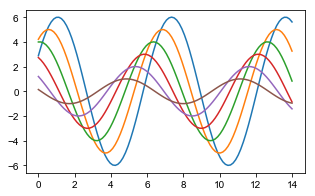绘制（二）set_style调用"dark"风格打印 sns.set_style(style=None, rc=None) style 风格，rc 线条设置
figure_new()
sns.set_style('dark') #设置打印风格
plt.title('seaborn-dark')
sinplot()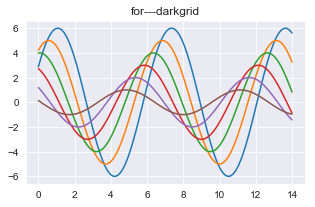绘制（三） with语句的绘制，域内使用风格，不改变整体的布局风格 with sns.axes_style(style=None, rc=None)
#sns.set() 初始化调色器
figure_new()
with sns.axes_style('whitegrid'): #使用with语句，定义域在域内使用
plt.title('seaborn-whitegrid-with-whitegrid')
sinplot()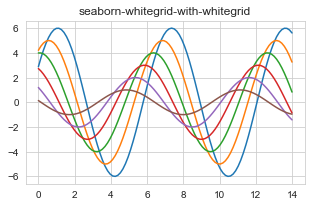绘制（四）for打印所有样式
style=['darkgrid', 'whitegrid', 'dark', 'white', 'ticks']
for i in range(5): #使用循环打印出5种风格
figure_new()
with sns.axes_style(style[i]):
plt.title('for---'+style[i])
sinplot()
#运行查看五种风格

despine 设置图型边框，轴线 despine(fig=None, ax=None, top=True, right=True, left=False, bottom=False, offset=None, trim=False)
plt.title('despine')
sinplot()
sns.despine(top=False,right=True,offset=20) #头部与右侧隐藏，offset图型偏移20
sns.set_style('white')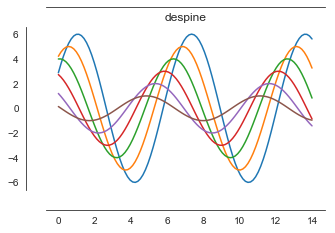绘制差异性子图
with sns.axes_style('whitegrid'): #绘制差异性子图
plt.subplot(211)
sinplot()
plt.subplot(212)
sns.set_context('notebook',font_scale=1.5,rc={'lines.linewidth':2.5}) #设置大小风格，font_scale字体大小，rc线宽
sns.set_style('ticks')
sns.despine()
sinplot()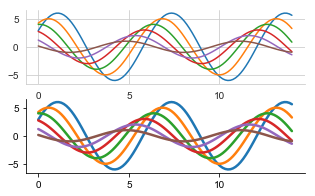2、探索分析图型
distplot单变量分析 distplot(a, bins=None, hist=True, kde=True, rug=False, fit=None, hist_kws=None, kde_kws=None, rug_kws=None, fit_kws=None, color=None, vertical=False, norm_hist=False, axlabel=None, label=None, ax=None) 柱型图
from scipy import stats
x=np.random.normal(size=100) #正态分布
sns.distplot(x,kde=True) #kde分布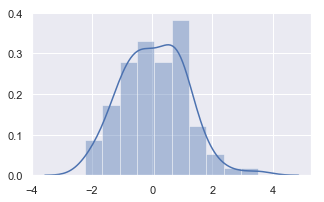概率密度分布
x=np.random.gamma(6,size=200)  #生成偏态分布数据集
sns.distplot(x,bins=20,kde=False,fit=stats.gamma) #fit似合形式scipy.stats概率密度函数指标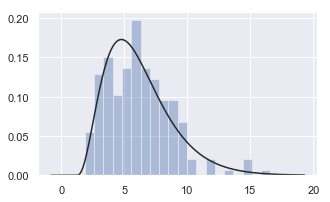jointplot二分类变量分析 jointplot(x, y, data=None, kind=‘scatter’, stat_func=None, color=None, height=6, ratio=5, space=0.2, dropna=True, xlim=None, ylim=None, joint_kws=None, marginal_kws=None, annot_kws=None, **kwargs)
散点图
mean,cov=[0,1],[(1,.5),(.5,1)] #均值，协方差
data=np.random.multivariate_normal(mean,cov,200) #指定均值与协方差生成随机数据
df=pd.DataFrame(data,columns=['x','y']) #生成DataFrame数据集
sns.jointplot(x='x',y='y',data=df)  #jointplot 散点图+单变量图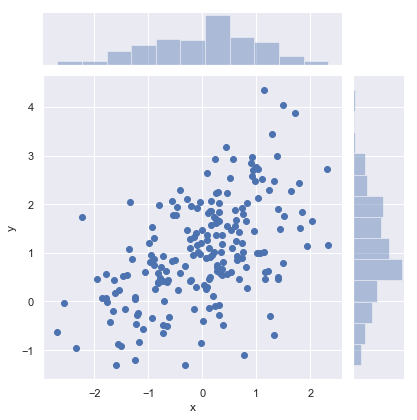大数据量，散点图色差化
x,y=np.random.multivariate_normal(mean,cov,1000).T #生成大数据量，横向数据集
with sns.axes_style('white'):
sns.jointplot(x=x,y=y,kind='hex',color='k') #kind=‘hex',数据按数量累计计算颜色深度，指定为黑色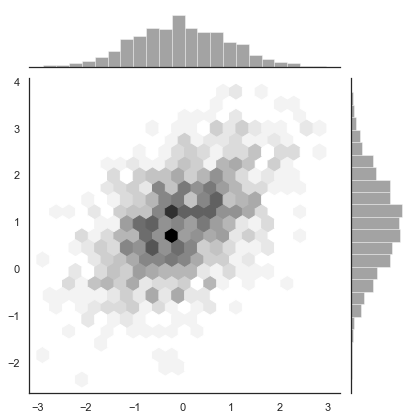3、多维度分析图
多维交叉散点图 pairplot(data, hue=None, hue_order=None, palette=None, vars=None, x_vars=None, y_vars=None, kind=‘scatter’, diag_kind=‘auto’, markers=None, height=2.5, aspect=1, dropna=True, plot_kws=None, diag_kws=None, grid_kws=None, size=None)
iris=sns.load_dataset('iris') #调用内置数据集
sns.pairplot(iris) #多维散点图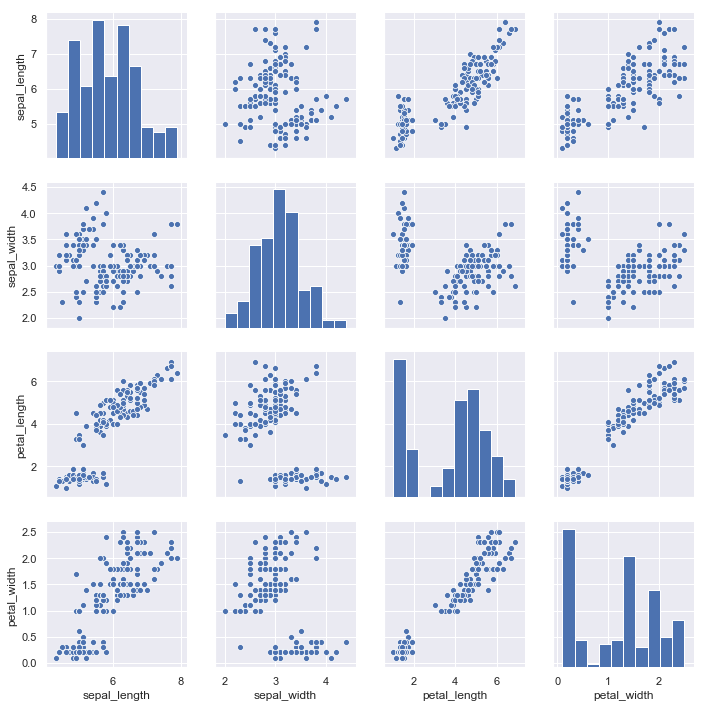4、回归分析
回归图 regplot(x, y, data=None, x_estimator=None, x_bins=None, x_ci=‘ci’, scatter=True, fit_reg=True, ci=95, n_boot=1000, units=None, order=1, logistic=False, lowess=False, robust=False, logx=False, x_partial=None, y_partial=None, truncate=False, dropna=True, x_jitter=None, y_jitter=None, label=None, color=None, marker=‘o’, scatter_kws=None, line_kws=None, ax=None)
tips=sns.load_dataset('tips') #调用小费数据集
plt.title('regplot')
sns.regplot(x='total_bill',y='tip',data=tips) #x,y轴定义，data数据集定义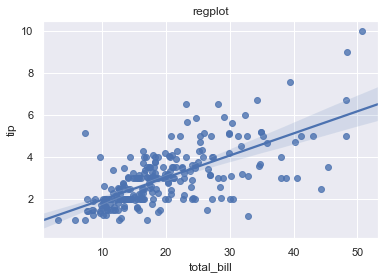多维交叉基点图
散点图交叉分析
tips=sns.load_dataset('tips')
sns.pairplot(tips[['total_bill','tip','sex']],hue='sex') #指定数据集，hue指定分类信息
plt.title('pairplot',fontdict={'fontsize':20},loc='left') #标题设定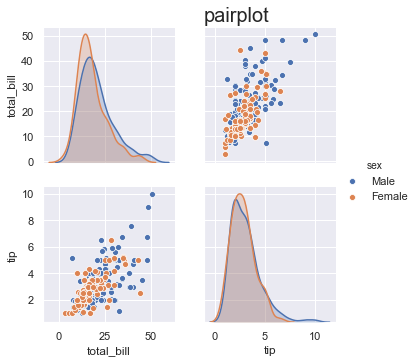分类数据集，回归分析
tips=sns.load_dataset('tips')
plt.title('regplot')
sns.regplot(x='size',y='tip',data=tips,x_jitter=.2) #离散型变量的回归分析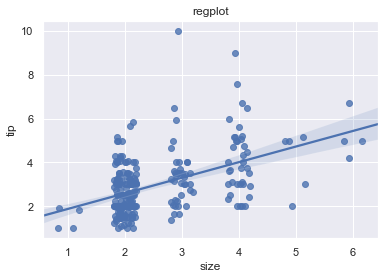stripplot 分类数 stripplot(x=None, y=None, hue=None, data=None, order=None, hue_order=None, jitter=True, dodge=False, orient=None, color=None, palette=None, size=5, edgecolor=‘gray’, linewidth=0, ax=None, **kwargs)
plt.title('stripplot-jitter')
sns.stripplot(x='day',y='total_bill',data=tips,jitter=True) #文本型分类，统计数据集,jitter重叠数据自动进行偏移(默认值True)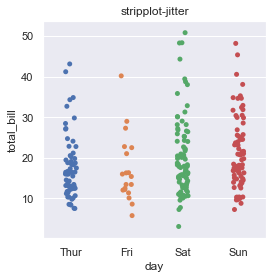分类数据的平行堆积 swarmplot(x=None, y=None, hue=None, data=None, order=None, hue_order=None, dodge=False, orient=None, color=None, palette=None, size=5, edgecolor=‘gray’, linewidth=0, ax=None, **kwargs)
plt.title('swarmplot')
sns.swarmplot(x='day',y='total_bill',data=tips)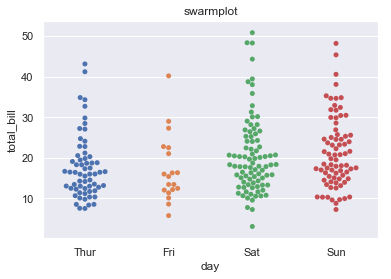箱图 boxplot(x=None, y=None, hue=None, data=None, order=None, hue_order=None, orient=None, color=None, palette=None, saturation=0.75, width=0.8, dodge=True, fliersize=5, linewidth=None, whis=1.5, notch=False, ax=None, **kwargs)
sns.boxplot(x='day',y='total_bill',hue='time',data=tips) #hue按颜色增加分类数据的箱型图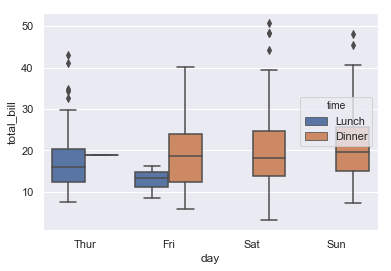使用split二分类字段数据组合
sns.violinplot(x='day',y='total_bill',hue='sex',data=tips,split=True)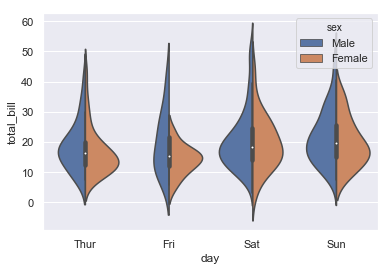inner 中心点设置，color颜色
sns.violinplot(x='day',y='total_bill',data=tips,inner=None,color='r')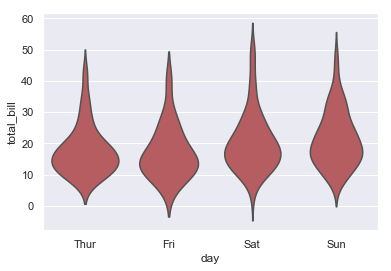fig,axes=plt.subplots(2,2)
sns.violinplot(x="day",y="total_bill",data=tips,inner="box",ax=axes[0,0])  #钢琴图内显示箱型图（左上）
sns.violinplot(x="day",y="total_bill",data=tips,inner="quartile",ax=axes[0,1])  #钢琴图内显示四分位数线（右上）
sns.violinplot(x="day",y="total_bill",data=tips,inner="point",ax=axes[1,0])  #钢琴图内显示具体数据点（左下）
sns.violinplot(x="day",y="total_bill",data=tips,inner="stick",ax=axes[1,1])  #钢琴图内显示具体数据棒（右下）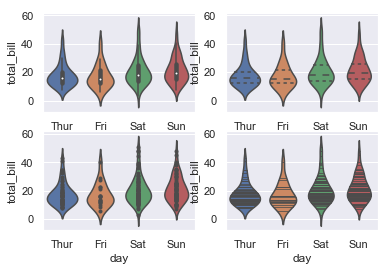调用调色板palette
planets = sns.load_dataset("planets")
ax = sns.violinplot(y="orbital_period", x="method",
data=planets[planets.orbital_period < 1000],
scale="width", palette="Set3")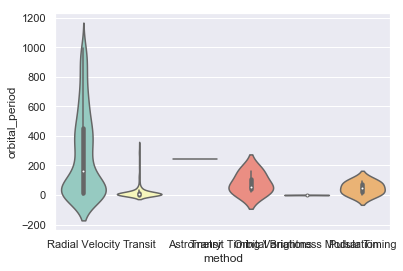图型包 调用matplotlib包的图型 factorplot(*args, **kwargs) 默认拆线图
差异图，数据的变化
sns.factorplot(x='day',y='total_bill',hue='smoker',data=tips) #不指定，拆线图

拆线图，kind定义图型类型
sns.factorplot(x='day',y='total_bill',hue='smoker',data=tips,kind='bar')

分类图，col定义图型分类
sns.factorplot(x='day',y='total_bill',hue='smoker',col='time',data=tips,kind='swarm')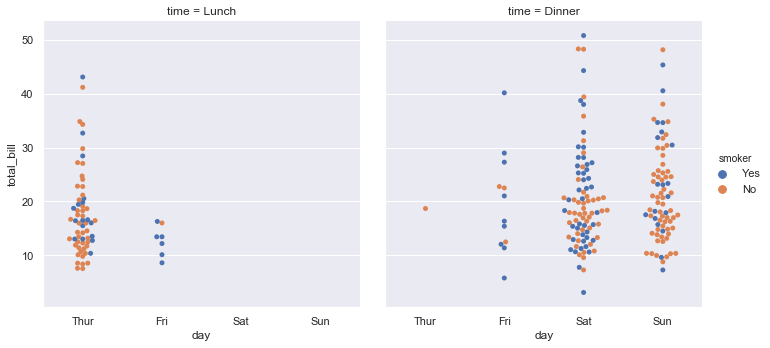箱型图，size定义大小，aspect图型长宽比
sns.factorplot(x='time',y='total_bill',hue='smoker',col='day',data=tips,kind='box',size=4,aspect=.5)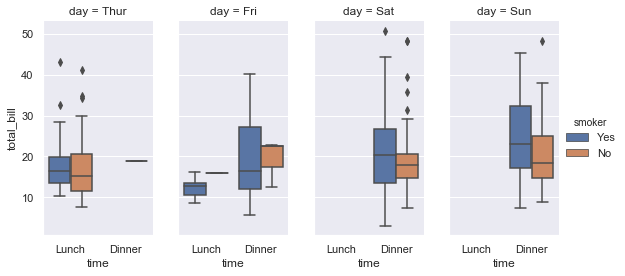子数据集的展示 组合型 FacetGrid(data, row=None, col=None, hue=None, col_wrap=None, sharex=True, sharey=True, height=3, aspect=1, palette=None, row_order=None, col_order=None, hue_order=None, hue_kws=None, dropna=True, legend_out=True, despine=True, margin_titles=False, xlim=None, ylim=None, subplot_kws=None, gridspec_kws=None, size=None)
分类子宽体图 g=.FacetGrid(数据集，分类） g.map(图型类别，字段）
柱型图
g=sns.FacetGrid(tips,col='time') #导入数据集，展示子集time(午餐，晚餐)
g.map(plt.hist,'tip') #调用图型,导入数据使用字段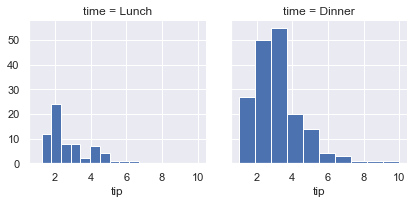散点图
g=sns.FacetGrid(tips,col='sex',hue='smoker')
g.map(plt.scatter,'total_bill','tip',alpha=.6) #alpha透明度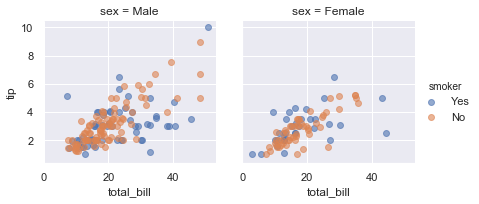交叉散点回归图
g=sns.FacetGrid(tips,row='smoker',col='time',margin_titles=True) #名称显示方式
g.map(sns.regplot,'size','total_bill',color='r',fit_reg=True,x_jitter=.7) #fig_reg回归线，x_jitter偏离大小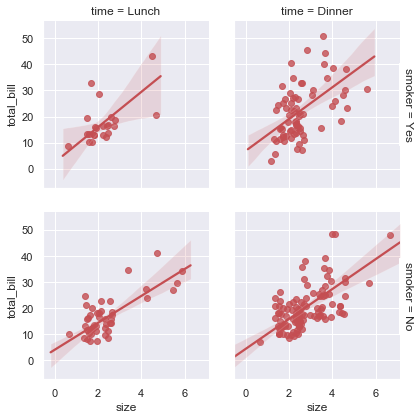分类柱型差值图
g=sns.FacetGrid(tips,col='day',size=4,aspect=.5) #指定大小，长宽比
g.map(sns.barplot,'sex','total_bill')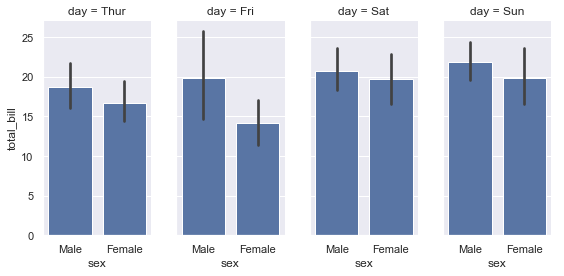#order_days=tips.day.values.categories #取出不重复列值，后续使用指定list进行排序
order_days=pd.Categorical(['Sun','Thur','Fri','Sat']) #指定列
g=sns.FacetGrid(tips,row='day',row_order=order_days,size=1.7,aspect=4.) #设置绘图顺序，指定图型大小，批定长宽比
g.map(sns.boxplot,'total_bill')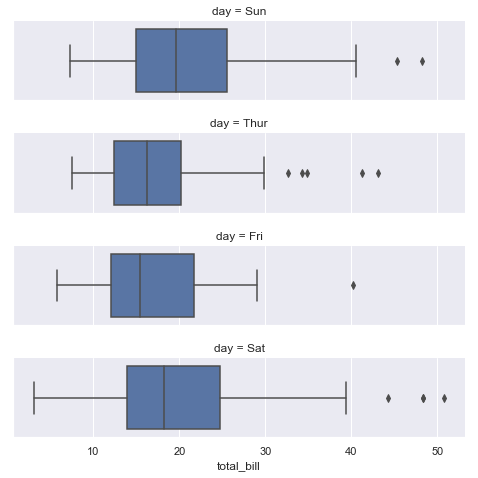pal=dict(Lunch='seagreen',Dinner='gray')
g=sns.FacetGrid(tips,hue='time',palette=pal,size=5)
g.map(plt.scatter,'total_bill','tip',s=50,alpha=.7,linewidth=.5,edgecolor='white') #s点大小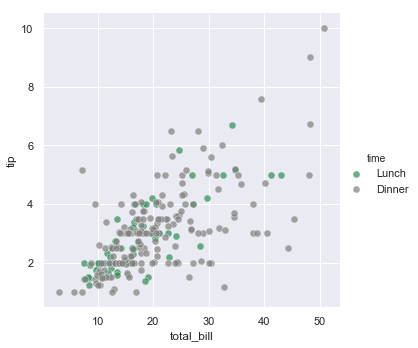g=sns.FacetGrid(tips,hue='sex',palette='Set1',size=5,hue_kws={'marker':["^",'v']})
g.map(plt.scatter,'total_bill','tip',s=100,linewidth=.5,edgecolor='white')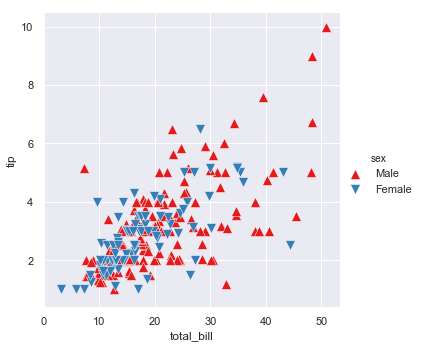多维度分类图型
with sns.axes_style('white'):
g=sns.FacetGrid(tips,row='sex',col='smoker',margin_titles=True,size=2.5)
g.map(plt.scatter,'total_bill','tip',color='#334488',edgecolor='white',lw=.5)
g.set_axis_labels('Total bill(US Dollars)','Tip') #轴标题
g.set(xticks=[10,30,50],yticks=[2,6,10]) #子图轴标签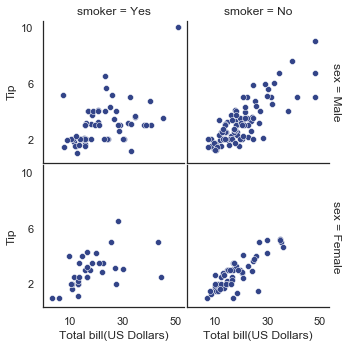多维分析图 PairGrid(data, hue=None, hue_order=None, palette=None, hue_kws=None, vars=None, x_vars=None, y_vars=None, diag_sharey=True, height=2.5, aspect=1, despine=True, dropna=True, size=None)
g = sns.PairGrid(iris, hue="species", palette="Set2",
hue_kws={"marker": ["o", "s", "D"]}) #palette色板,marker标记形状
g = g.map(plt.scatter, linewidths=1, edgecolor="w", s=40)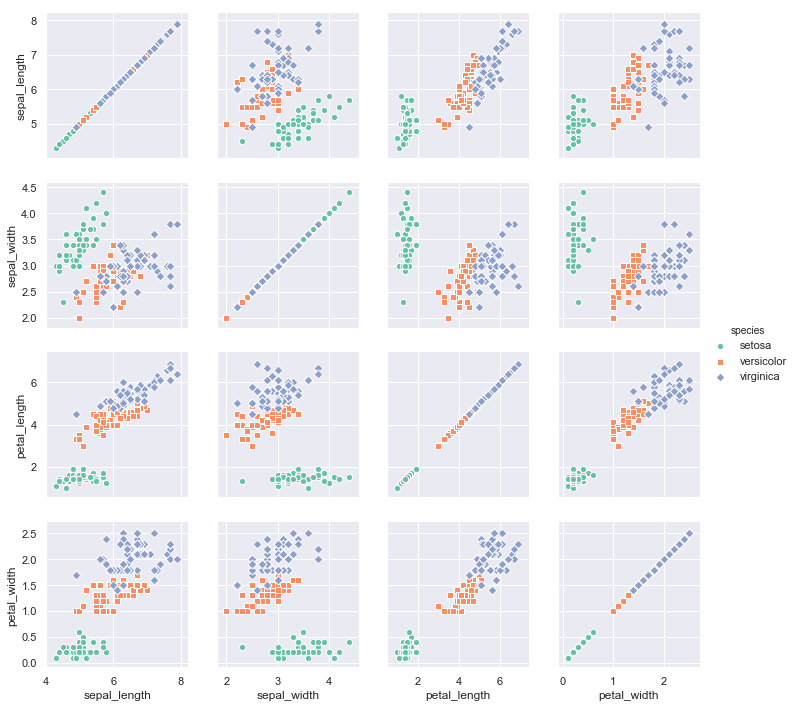iris=sns.load_dataset('iris')

g = sns.PairGrid(iris)
g = g.map_upper(plt.scatter) #高位散点图
g = g.map_lower(sns.kdeplot, cmap="Blues_d") #低位核密度图
g = g.map_diag(sns.kdeplot, lw=3, legend=False)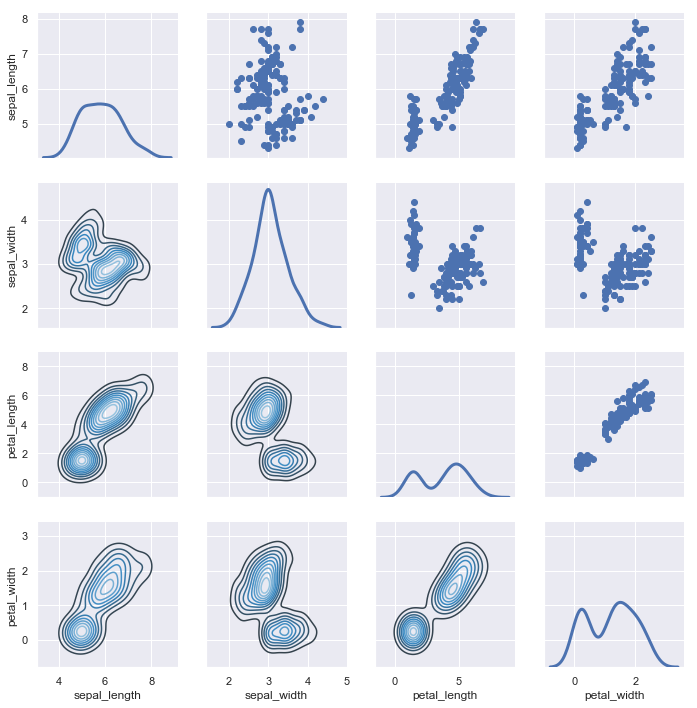iris=sns.load_dataset('iris')
g = sns.PairGrid(iris, hue="species")
g = g.map_diag(plt.hist, histtype="step", linewidth=3) #histtype设置柱型样式，linewidth线宽
g = g.map_offdiag(plt.scatter)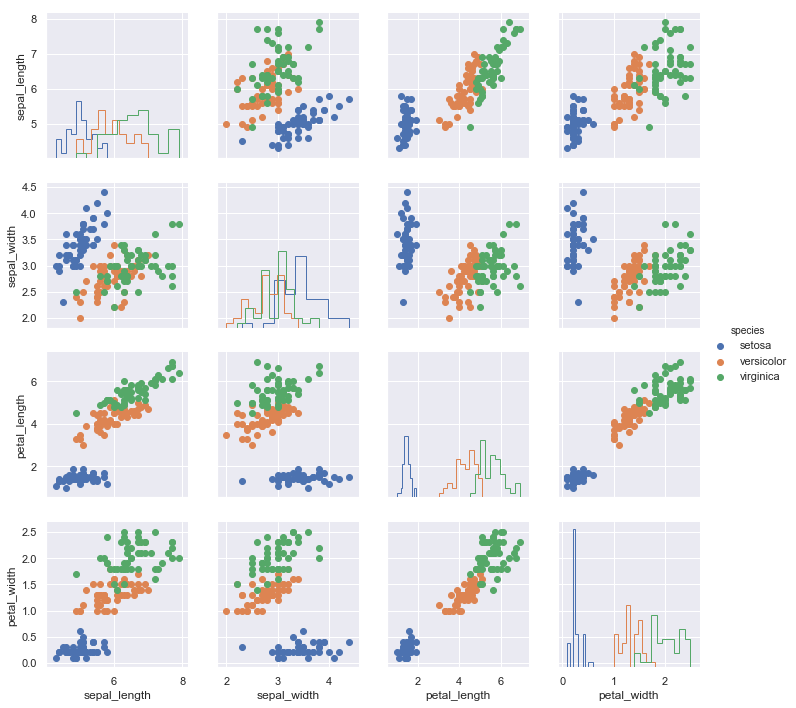热力图
np.random.seed(0)
df=np.random.rand(3,3) #生成矩阵数据
sns.heatmap(df)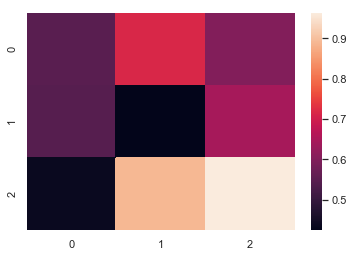设置最大最小值，设置中心点
np.random.seed(0) #设置时钟起始值
df=np.arange(25).reshape(5,5) #生成矩阵
sns.heatmap(df,vmin=5,vmax=20,center=15) #vmin,vmax设置色板的最大最小值，center设置中心值，上下按颜色区分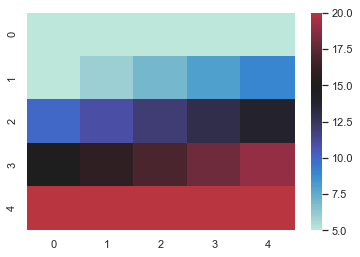热力图默认参数
figure_new()
flights=flights.pivot('month','year','passengers')
ax=sns.heatmap(flights,cbar=False) #隐藏色度条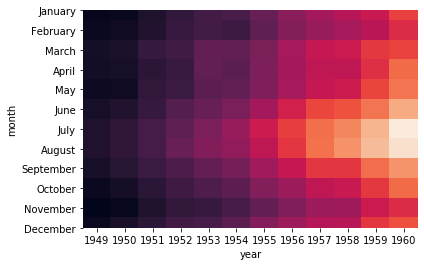热力图，色板放到x轴
flights = sns.load_dataset("flights") #调用航班数据
flights = flights.pivot("month", "year", "passengers") #交叉表
grid_kws = {"height_ratios": (.9, .05), "hspace": 0.4} #定义图型大小，height_rations 高度0.9，和高度，0.05，hspace间隔设置
f, (ax, cbar_ax) = plt.subplots(2, gridspec_kw=grid_kws) #绘制图型框架，定义ax,cbar_ax框大小
ax = sns.heatmap(flights, ax=ax,
cbar_ax=cbar_ax,
cbar_kws={"orientation": "horizontal"}) #图型加载到框架内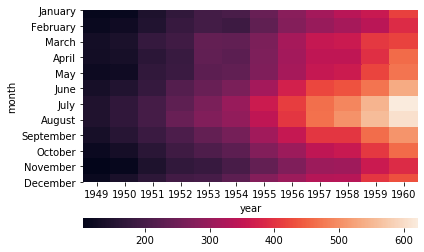corr=np.corrcoef(np.random.randn(10,200))
with sns.axes_style('white'):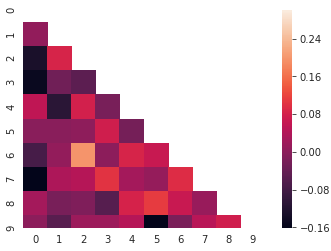展开全文• matplotlib是基于Python的绘图库，广泛用于Python科学计算界。它完整支持二维绘图以及部分支持三维绘图。该绘图库致力于能适应广泛的用户需求。它可以根据所选的用户接口工具来嵌入绘图算法。与此同时，对于使用GTK+...
matplotlib是基于Python的绘图库，广泛用于Python科学计算界。它完整支持二维绘图以及部分支持三维绘图。该绘图库致力于能适应广泛的用户需求。它可以根据所选的用户接口工具来嵌入绘图算法。与此同时，对于使用GTK+、Qt、Tk、FLTK、wxWidgets与Cocoa的所有主要桌面操作系统，matplotlib能支持交互式绘图。在Python的交互式shell中，我们可以使用简单的、过程式的命令交互式地调用matplotlib来生成图形，与使用Mathematica、IDL或者MATLAB绘图非常相似。matplotlib也可以嵌入到无报文头的Web服务器中，以提供基于光栅（如PNG格式）与向量（如Postscript、PDF以及纸面效果很好的SVG格式）这两种格式的图形硬拷贝。
一、历史溯源—— 硬件锁问题
我们其中一位开发者（John Hunter）与他的研究癫痫症的同事们试图在不借助专有软件的情况下进行脑皮层电图（ECoG）分析，于是便有了最初的matplotlib。John Hunter当时所在的实验室只有一份电图分析软件的许可证，但有各式各样的工作人员，如研究生、医科学生、博士后、实习生、以及研究员，他们轮流共享该专有软件的硬件电子锁。生物医学界广泛使用MATLAB进行数据分析与可视化，所以Hunter着手使用基于MATLAB的matplotlib来代替专有软件，这样很多研究员都可以使用并且对其进行扩展。但是MATLAB天生将数据当作浮点数的数组来处理。然而在实际情况中，癫痫手术患者的医疗记录具有多种数据形式（CT、MRI、ECoG与EEG等），并且存储在不同的服务器上。MATLAB作为数据管理系统勉强能应付这样的复杂性。由于感到MATLAB不适合于这项任务，Hunter开始编写一个新的建立在用户接口工具GTK+（当时是Linux下的主流桌面视窗系统）之上的Python应用程序。
所以matplotlib这一GTK+应用程序最初便被开发成EEG/ECoG可视化工具。这样的用例决定了它最初的软件架构。matplotlib最初的设计也服务于另一个目的：代替命令驱动的交互式图形生成（这一点MATLAB做得很好）工具。MATLAB的设计方法使得加载数据文件与绘图这样的任务非常简单，而要使用完全面向对象的API则会在语法上过于繁琐。所以matplotlib也提供状态化的脚本编程接口来快速、简单地生成与MATLAB类似的图形。因为matplotlib是Python库，所以用户可以使用Python中各种丰富的数据结构，如列表、辞典与集合等等。二、 matplotlib软件架构概述
顶层的matplotlib对象名为Figure，它包含与管理某个图形的所有元素。matplotlib必须完成的一个核心架构性任务是实现Figure的绘制与操作框架，并且做到该框架与Figure到用户视窗接口或硬拷贝渲染行为是分离的。这使得我们可以为Figure添加越来越复杂的特性与逻辑，同时保持“后端”或输出设备的相对简化。matplotlib不仅封装了用于向多种设备渲染的绘图接口，还封装了基本事件处理以及多数流行的用户界面工具的视窗功能。因此，用户可以创建相当丰富的交互式图形算法与用户界面工具（用到可能存在的鼠标与键盘），而又不必修改matplotlib已经支持的6种界面工具。
要实现这些，matplotlib的架构被逻辑性地分为三层。这三层逻辑可以视为一个栈。每层逻辑知道如何与其下的一层逻辑进行通信，但在下层逻辑看来，上层是透明的。这三层从底向上分别为：后端、美工与脚本。

1、 后端
matplotlib逻辑栈最底层是后端，它具体实现了下面的抽象接口类：
FigureCanvas对绘图表面（如“绘图纸”）的概念进行封装。Renderer执行绘图动作（如“画笔”）。Event处理键盘与鼠标事件这样的用户输入。
对于如Qt这样的用户界面工具，FigureCanvas中包含的具体实现可以完成三个任务：将自身嵌入到原生的Qt视窗（QtGui.QMainWindow）中，能将matplotlib的Renderer命令转换到canvas上（QtGui.QPainter），以及将原生Qt事件转换到matplotlib的Event框架下（后者产生回调信号让上行监听者进行处理）。抽象基类定义在matplotlib.backend_bases中，且所有派生类都定义在如matplotlib.backends.backend_qt4agg这样的专用模块中。对于专门生成硬拷贝输出（如PDF、PNG、SVG或PS）的纯图像后端而言，FigureCanvas的实现可能只是简单地建立一个类似文件的对象，其中定义默认的文件头、字体与宏函数，以及Renderer创建的个别对象（如直线、文本与矩形等）。
Renderer的任务是提供底层的绘图接口，即在画布上绘图的动作。上文已经提到，最初的matplotlib程序是一个基于GTK+的ECoG查看器，而且很多早期设计灵感都源自当时已有的GDK/GTK+的API。最初Renderer的API源自GDK的Drawable接口，后者实现了draw_point、draw_line、draw_rectangle、draw_image、draw_polygon以及draw_glyphs这样的基本方法。我们完成的每个不同后端——最早有PostScript与GD——都实现了GDK的Drawable，并将其转换为独立于后端的原生绘图命令。如上所述，这毫无必要地增加了后端的实现复杂度，原因是单独实现Drawable造成函数泛滥。此后，Renderer已经被极大的简化，将matplotlib移植到新的用户界面或文件格式已经是非常简单的过程。
一个对matplotlib有利的设计决定是支持使用C++模板库Anti-Grain Geometry（缩写为agg[She06]）的基于像素点的核心渲染器。这是一个高性能库，可以进行2D反锯齿渲染，生成的图像非常漂亮。matplotlib支持将agg后端渲染的像素缓存插入到每种支持的用户界面中，所以在不同的UI与操作系统下都能得到精确像素点的图形。因为matplotlib生成的PNG输出也使用agg渲染器，所以硬拷贝与屏幕显示完全相同，也就是说在不同的UI与操作系统下，PNG的输出所见即所得。
matplotlib的Event框架将key-press-event或mouse-motion-event这样的潜在UI事件映射到KeyEvent或MouseEvent类。用户可以连接到这些事件进行函数回调，以及图形与数据的交互，如要pick一个或一组数据点，或对图形或其元素的某方面性质进行操作。下面的示例代码演示了当用户键入‘t’时，对Axes窗口中的线段进行显示开关。
import numpy as np
import matplotlib.pyplot as plt

def on_press(event):
if event.inaxes is None: return
for line in event.inaxes.lines:
if event.key=='t':
visible = line.get_visible()
line.set_visible(not visible)
event.inaxes.figure.canvas.draw()

fig, ax = plt.subplots(1)

fig.canvas.mpl_connect('key_press_event', on_press)

ax.plot(np.random.rand(2, 20))

plt.show()

对底层UI事件框架的抽象使得matplotlib的开发者与最终用户都可以编写UI事件处理代码，而且“一次编写，随处运行”。譬如，在所有用户界面下都可以对matplotlib图像进行交互式平移与放缩，这种交互式操作就是在matplotlib的事件框架下实现的。
2 、Artis层
（称之为“ 美工”其实是一个汉化的形象说法，因为“ 在哪里画，用什么画，画什么图？ ”这不正是美工的工作么？）
Artist层次结构处于matplotlib的中间层，负责很大一部分繁重的计算任务。延续之前将后端的FigureCanvas看作画纸的比喻，Artis对象知道如何用Renderer（画笔）在画布上画出墨迹。matplotlib中的Figure就是一个Artist对象实例。标题、直线、刻度标记以及图像等等都对应某个Artist实例（如图11.3）。Artist的基类是matplotlib.artist.Artist，其中包含所有Artist的共享属性，包括从美工坐标系统到画布坐标系统的变换（后面将详细介绍）、可见性、定义用户可绘制区域的剪切板、标签，以及处理“选中”这样的用户交互动作的接口，即在美工层检测鼠标点击事件。Artist层于后端之间的耦合性存在于draw方法中。譬如，下面假想的SomeArtist类是Artist的子类，它要实现的关键方法是draw，用来传递给后端的渲染器。Artist不知道渲染器要向哪种后端进行绘制（PDF、SVG与GTK+绘图区等），但知道Renderer的API，并且会调用适当的方法（draw_text或draw_path）。因为Renderer能访问画布，并且知道如何绘制，所以draw方法将Artist的抽象表示转换为像素缓存中的颜色、SVG文件中的轨迹或者其他具体表示。
class SomeArtist(Artist):
'An example Artist that implements the draw method'

def draw(self, renderer):
"""Call the appropriate renderer methods to paint self onto canvas"""
if not self.get_visible():  return

# create some objects and use renderer to draw self here
renderer.draw_path(graphics_context, path, transform)

该层次结构中有两种类型的Artist。基本Artist表示我们在图形中能看到的一类对象，如Line2D、Rectangle、Circle与Text。复合Artist是Artist的集合，如Axis、Tick、Axes与Figure。每个复合Artsit可能包含其他复合Artist与基本Artist。譬如，Figure包含一个或多个Axes，并且Figure的背景是基本的Rectangle。
最重要的复合Artist是Axes，其中定义了大多数matplot的绘图方法。Axes不仅仅包含大多数构成绘图背景（如标记、轴线、网格线、色块等）的图形元素，还包括了大量生成基本Artist并添加到Axes实例中的帮助函数。譬如，表11.1列出了一些Axes函数，这些函数进行对象的绘制，并将它们存储在Axes实例中。
表11.1：Axes的方法样例及其创建的Artist实例
| 方法 | 创建对象 | 存储位置 | | Axes.imshow | 一到多个matplotlib.image.AxesImage | Axes.images | | Axes.hist | 大量matplotlib.patch.Rectangle | Axes.patches | | Axes.plot | 一到多个matplotlib.lines.Line2D | Axes.lines |
下面这个简单的Python脚本解释了以上架构。它定义了后端，将Figure链接至该后端，然后使用数组库numpy创建10,000个正太分布的随机数，最后绘制出它们的柱状图。
# Import the FigureCanvas from the backend of your choice
#  and attach the Figure artist to it.
from matplotlib.backends.backend_agg import FigureCanvasAgg as FigureCanvas
from matplotlib.figure import Figure
fig = Figure()
canvas = FigureCanvas(fig)

# Import the numpy library to generate the random numbers.
import numpy as np
x = np.random.randn(10000)

# Now use a figure method to create an Axes artist; the Axes artist is
#  added automatically to the figure container fig.axes.
# Here "111" is from the MATLAB convention: create a grid with 1 row and 1
#  column, and use the first cell in that grid for the location of the new
#  Axes.

# Call the Axes method hist to generate the histogram; hist creates a
#  sequence of Rectangle artists for each histogram bar and adds them
#  to the Axes container.  Here "100" means create 100 bins.
ax.hist(x, 100)

# Decorate the figure with a title and save it.
ax.set_title('Normal distribution with $\mu=0, \sigma=1$')
fig.savefig('matplotlib_histogram.png')

3 、脚本层（pyplot）
使用以上API的脚本效果很好，尤其是对于程序员而言，并且在编写Web应用服务器、UI应用程序或者是与其他开发人员共享的脚本时，这通常是比较合适的编程范式。对于日常用途，尤其对于非专业程序员而要完成一些交互式的研究工作的实验科学家而言，以上API的语法可能有些难以掌握。大多数用于数据分析与可视化的专用语言都会提供轻量级的脚本接口来简化一些常见任务。matplotlib在其matplotlib.pyplot接口中便实现了这一点。以上代码改用pyplot之后如下所示。
import matplotlib.pyplot as plt
import numpy as np

x = np.random.randn(10000)
plt.hist(x, 100)
plt.title(r'Normal distribution with $\mu=0, \sigma=1$')
plt.savefig('matplotlib_histogram.png')
plt.show()图11.4：用pyplot绘制的柱状图
pyplot是一个状态化接口，大部分工作是处理样本文件的图形与坐标的生成，以及与所选后端的连接。它还维护了模块级的内部数据结构。这些数据结构表示了直接接收绘图命令的当前图形与坐标
下面仔细分析示例脚本中比较重要的几行，观察其内部状态的管理方式。
import matplotlib.pyplot as plt：当pyplot模块被加载时，它分析本地配置文件。配置文件除了完成一些其他工作外，主要声明了默认的后端。可能是类似QtAgg的用户接口后端，于是上面的脚本将导入GUI框架并启动嵌入了图形的Qt窗口；或者可以是一个类似Agg的纯图像后端，这样脚本会生成硬拷贝输出然后退出。plt.hist(x, 100)：这是脚本中第一个绘图命令。pyplot会检测其内部数据结构已查看是否存在当前Figure实例。如果存在，则提取当前Axes，并将绘图行为导向Axes.hist的API调用。在该脚本中不存在Figure实例，所以会生成一个FIgure与Axes，并将它们设为当前值，然后将绘图行为导向Axes.hist。 plt.hist(x, 100): This is the first plotting command in the script. pyplot will check its internal data structures to see if there is a current Figure instance. If so, it will extract the current Axes and direct plotting to the Axes.hist API call. In this case there is none, so it will create a Figure and Axes, set these as current, and direct the plotting to Axes.hist. plt.title(r'Normal distribution with $\mu=0, \sigma=1$'): As above, pyplot will look to see if there is a current Figure and Axes. Finding that there is, it will not create new instances but will direct the call to the existing Axes instance method Axes.set_title. plt.show(): This will force the Figure to render, and if the user has indicated a default GUI backend in their configuration file, will start the GUI mainloop and raise any figures created to the screen.
三、总结
1、matplotlib是一个非常庞大的系统，而且从诞生到现在繁荣发展经历了几十年的时间，里面所涉及到的软件架构很是复杂。
2、matplotlib的使用可以从三个层面上去入手，即“后端——美工——脚本”。
（1）后端：我们当然可以从最底层的后端搭建一个图形，负责软件与绘图硬件的交互，图形的各种渲染等等，这异常困难，而且对于程序员的开发效率来说，十分缓慢，因为绘图的框架整个都要自己去搭建，很麻烦。
（2）美工：这是在后端的基础之上的进一步封装，有一点类似于GDI+绘图方式，我们只关心“ 在哪里画 、用什么笔、 画什么图 ”，所用的笔，所用的颜料，画的图形类型（折线图，条形图，散点图等等）这些已经封装好了，封装是通过一系列的类去完成的。
（3）脚本：脚本是matplotlib的更高层封装，也就是我们平时所用的pyplot模块的高级封装，使用脚本，我们再也不用关心这个图形到底是怎么画的了，我们只需要关心画图的效果即可。适用“ 后端 ”、“ 美工 ”、要很多代码才能完成的事，使用脚本一句话就可以实现，如：
plt.plot(x,y,color='red',linewidth=3,linestyle='--')
就是这么简单的一句话如果要自己从头搭建，是一件很困难的事情。
展开全文• 一 Python常用扩展 二 Numpy科学计算 三 Pandas数据分析Matplotlib画图


目录(?)[+]
一 Python常用扩展包二 Numpy科学计算包三 Pandas数据分析包四 Matplotlib画图包

前面几篇文章采用的案例的方法进行介绍的，这篇文章主要介绍Python常用的扩展包，同时结合数据挖掘相关知识介绍该包具体的用法，主要介绍Numpy、Pandas和Matplotlib三个包。目录：        一.python常用扩展包        二.Numpy科学计算包        三.Pandas数据分析包        四.Matplotlib绘图包
前文推荐：       【Python数据挖掘课程】一.安装Python及爬虫入门介绍       【Python数据挖掘课程】二.Kmeans聚类数据分析及Anaconda介绍       【Python数据挖掘课程】三.Kmeans聚类代码实现、作业及优化       【Python数据挖掘课程】四.决策树DTC数据分析及鸢尾数据集分析       【Python数据挖掘课程】五.线性回归知识及预测糖尿病实例        绘图强推：http://python.jobbole.com/85106/
希望这篇文章对你有所帮助，尤其是刚刚接触数据挖掘以及大数据的同学，这些基础知识真的非常重要。如果文章中存在不足或错误的地方，还请海涵~        部分截图参考张良均的《Python数据分析与挖掘实战》，推荐大家购买阅读。

一. Python常用扩展包
参考张良均的《Python数据分析与挖掘实战》，下图展示了常见的Python扩展包。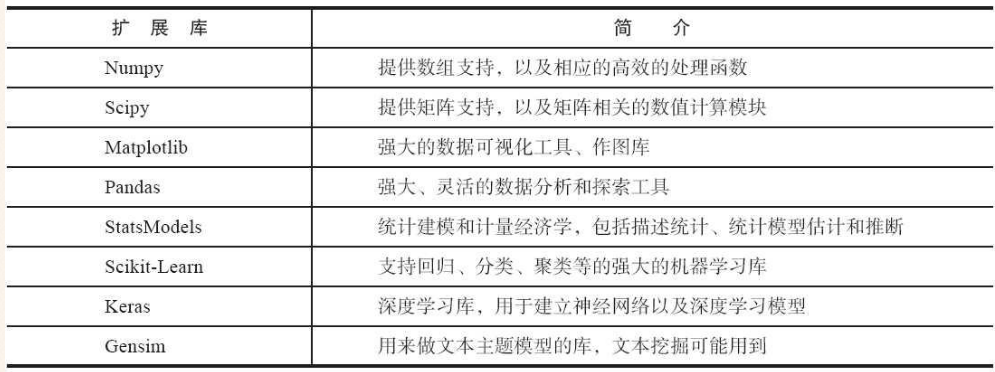常用的包主要包括：        1.Numpy        Python没有提供数组，列表（List）可以完成数组，但不是真正的数据，当数据量增大时，，它的速度很慢。所以Numpy扩展包提供了数组支持，同时很多高级扩展包依赖它。例如：Scipy、Matplotlib、Pandas。
2.Scipy        该包提供矩阵支持，以及矩阵相关的数值计算模块。如果说Numpy让Python有了Matlab的味道，那么Scipy就让Python真正地成为二半个Matlib。因为涉及到矩阵内容，而课程中主要使用数组，所以不再介绍。
3.Pandas        Pandas是面板数据（Panel Data）的简写。它是Python最强大的数据分析和探索工具，因金融数据分析工具而开发，支持类似SQL的数据增删改查，支持时间序列分析，灵活处理缺失数据，后面详细介绍。
4.Scikit-Learn        Scikit-Learn是一个基于python的用于数据挖掘和数据分析的简单且有效的工具，它的基本功能主要被分为六个部分：分类(Classification)、回归(Regression)、聚类(Clustering)、数据降维(Dimensionality Reduction)、模型选择(Model Selection)、数据预处理(Preprocessing)，前面写的很多文章算法都是出自该扩展包。        详见官网：http://scikit-learn.org/stable/
5.Matplotlib        该包主要用于绘图和绘表，强大的数据可视化工具，做图库，语法类似MATLAB。同时，Seaborn也是数据可视化的工具包。        注意：这些包在Anaconda集成环境中已经存在，可以直接使用，最早我是通过Python2.7来编写代码的，安装过程通过pip install numpy，而且安装顺序非常讲究，容易出错，所以推荐大家使用该集成包。
二. Numpy科学计算包
NumPy（Numeric Python）系统是Python的一种开源的数值计算扩展，一个用python实现的科学计算包。它提供了许多高级的数值编程工具，如：矩阵数据类型、矢量处理，以及精密的运算库。专为进行严格的数字处理而产生。        推荐学习：http://old.sebug.net/paper/books/scipydoc/numpy_intro.html        下面通过这段代码详细讲解这个包在数据分析中的常见用法：        1.一维数组处理

[python]
view plain
copy

print
?

#导入包并重命名  import numpy as np    #定义一维数组  a = np.array([2, 0, 1, 5, 8, 3])  print u‘原始数据:’, a    #输出最大、最小值及形状  print u‘最小值:’, a.min()  print u‘最大值:’, a.max()  print u‘形状’, a.shape    #数据切片  print u‘切片操作:’  print a[:-2]  print a[-2:]  print a[:1]    #排序  print type(a)  a.sort()  print u‘排序后:’, a

#导入包并重命名
import numpy as np


#定义一维数组 a = np.array([2, 0, 1, 5, 8, 3]) print u'原始数据:', a #输出最大、最小值及形状 print u'最小值:', a.min() print u'最大值:', a.max() print u'形状', a.shape #数据切片 print u'切片操作:' print a[:-2] print a[-2:] print a[:1] #排序 print type(a) a.sort() print u'排序后:', a
输出结果如下所示：

[html]
view plain
copy

print
?

原始数据: [2 0 1 5 8 3]  最小值: 0  最大值: 8  形状 (6L,)  切片操作:  [2 0 1 5]  [8 3]    <type ‘numpy.ndarray’>  排序后: [0 1 2 3 5 8]

原始数据: [2 0 1 5 8 3]
最小值: 0
最大值: 8
形状 (6L,)
切片操作:
[2 0 1 5]
[8 3]

<type 'numpy.ndarray'>
排序后: [0 1 2 3 5 8]
核心代码：        代码通过np.array定义了一个数组[2, 0, 1, 5, 8, 3]，其中min计算最小值，max计算最大值，shape表示数组的形状，因为是一维数组，故6L（6个数字）。        最重要的一个知识点是数组的切片操作，因为在数据分析过程中，通常会对数据集进行”80%-20%”或”70%-30%”的训练集和测试集划分，通常采用的方法就是切片。        a[:-2]表示从头开始获取，”-2”表示后面两个值不取，结果：[2 0 1 5]        a[-2:]表示后往前数两个数字，获取数字至结尾，即获取最后两个值[8 3]        a[:1]表示从头开始获取，获取1个数字，即        2.二维数组处理        注意的是定义二维数组括号不要弄错，正确的应该是：[[1,2,3],[4,5,6]]        同时计算机的存储下标都是从0开始计算的。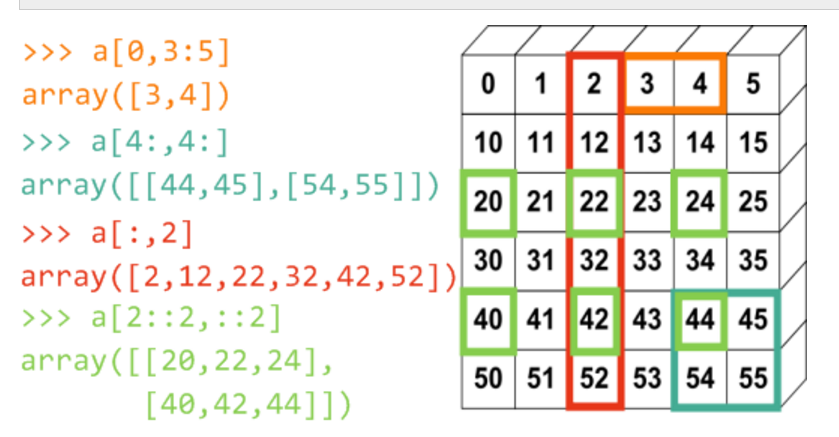代码如下：

[python]
view plain
copy

print
?

#定义二维数组  import numpy as np  c = np.array([[1, 2, 3, 4],[4, 5, 6, 7], [7, 8, 9, 10]])    #获取值  print u‘形状:’, c.shape  print u‘获取值:’, c  print u‘获取某行:’  print c[:]  print u‘获取某行并切片:’  print c[:-1]  print c[-1:]    #获取具体某列值  print u‘获取第3列:’  print c[:,np.newaxis, 2]    #调用sin函数  print np.sin(np.pi/6)  print type(np.sin(0.5))    #范围定义  print np.arange(0,4)  print type(np.arange(0,4))

#定义二维数组
import numpy as np
c = np.array([[1, 2, 3, 4],[4, 5, 6, 7], [7, 8, 9, 10]])

 #获取值 print u'形状:', c.shape print u'获取值:', c print u'获取某行:' print c[:] print u'获取某行并切片:' print c[:-1] print c[-1:] #获取具体某列值 print u'获取第3列:' print c[:,np.newaxis, 2] #调用sin函数 print np.sin(np.pi/6) print type(np.sin(0.5)) #范围定义 print np.arange(0,4) print type(np.arange(0,4))
代码输出结果如下所示：

[python]
view plain
copy

print
?

形状: (3L, 4L)  获取值: 4  获取某行:  [4 5 6 7]  获取某行并切片:  [1 2 3]    获取第3列:  [      ]  0.5  <type ’numpy.float64’>  [0 1 2 3]  <type ’numpy.ndarray’>

形状: (3L, 4L)
获取值: 4
获取某行:
[4 5 6 7]
获取某行并切片:
[1 2 3]

获取第3列:
[

]
0.5
<type 'numpy.float64'>
[0 1 2 3]
<type 'numpy.ndarray'>
需要注意：       （1）获取二维数组中的某行，如第2行数据[4,5,6,7]，采用方法是：c[:]；       （2）获取二维数组中的某列，如第2列数据[  ]，c[:,np.newaxis, 2]。因为通常在数据可视化中采用获取某列数据作为x或y坐标，同时多维数据也可以采用PCA降低成两维数据，再进行显示。        最后希望读者自己去阅读该段代码。

三. Pandas数据分析包
Pandas是面板数据（Panel Data）的简写。它是Python最强大的数据分析和探索工具，因金融数据分析工具而开发，支持类似SQL的数据增删改查，支持时间序列分析，灵活处理缺失数据。        注意：首先声明改包功能非常强大，我只是学习了它的非常小的一部分，后面随着学习深入会写更多它的用法，同时建议读者自行学习，不喜勿喷。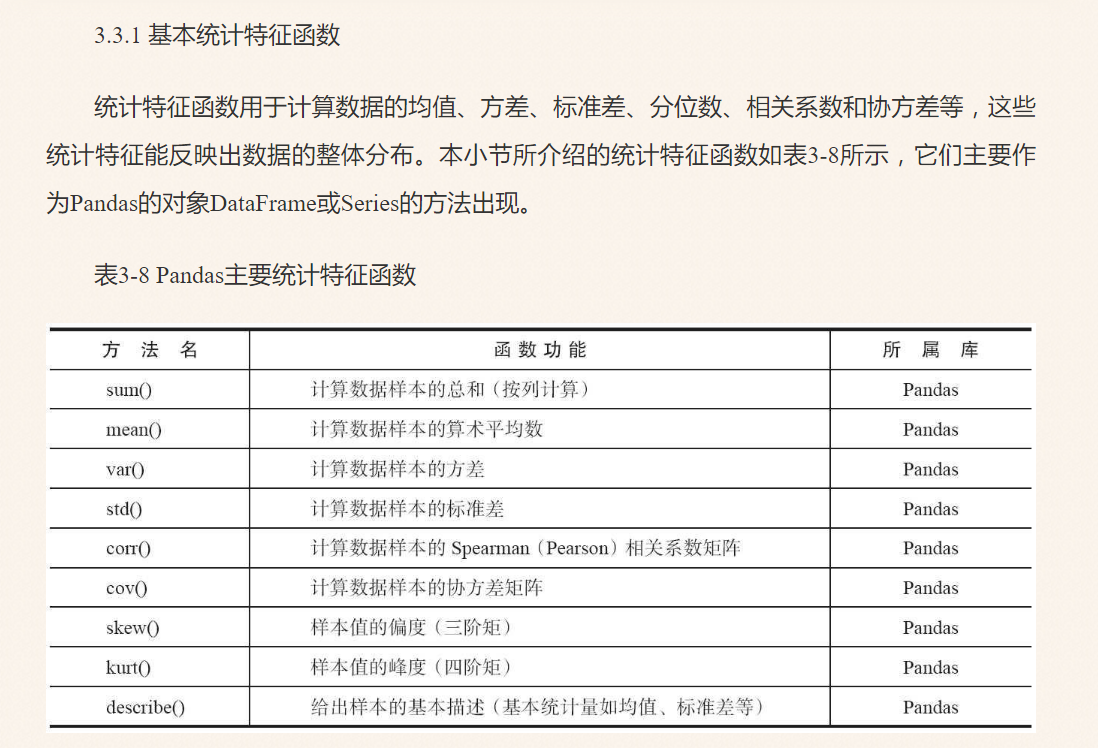约定俗成的导入惯例：        from pandas import Series, DataFrame        import pandas as pd
1.常见用法：读写文件        这里读文件最常用的是两种方法：

[python]
view plain
copy

print
?

#写入excel文件：
df.to_excel('foo.xlsx', sheet_name='Sheet1')

 #从excel文件中读取： pd.read_excel('foo.xlsx', 'Sheet1', index_col=None, na_values=['NA']) #写入csv文件： df.to_csv('foo.csv') #从csv文件中读取： pd.read_csv('foo.csv') #写入HDF5存储： df.to_hdf('foo.h5','df') #从HDF5存储中读取： pd.read_hdf('foo.h5','df')
下面通过一个具体的案例来讲解该包，这里读取的数据是张良均的《Python数据分析与挖掘实战》的第六章的电力用户数据集，missing_data.xls文件。内容如下，共3列数据，分别是用户A、用户B、用户C，共21行，对应21天的用电量，其中包含缺失值。

[python]
view plain
copy

print
?

235.8333    324.0343    478.3231  236.2708    325.6379    515.4564  238.0521    328.0897    517.0909  235.9063        514.89  236.7604    268.8324          404.048 486.0912  237.4167    391.2652    516.233  238.6563    380.8241      237.6042    388.023 435.3508  238.0313    206.4349    487.675  235.0729          235.5313    400.0787    660.2347      411.2069    621.2346  234.4688    395.2343    611.3408  235.5   344.8221    643.0863  235.6354    385.6432    642.3482  234.5521    401.6234      236 409.6489    602.9347  235.2396    416.8795    589.3457  235.4896        556.3452  236.9688        538.347

235.8333  324.0343    478.3231
236.2708    325.6379    515.4564
238.0521    328.0897    517.0909
235.9063        514.89
236.7604    268.8324
404.048 486.0912
237.4167    391.2652    516.233
238.6563    380.8241
237.6042    388.023 435.3508
238.0313    206.4349    487.675
235.0729
235.5313    400.0787    660.2347
411.2069    621.2346
234.4688    395.2343    611.3408
235.5   344.8221    643.0863
235.6354    385.6432    642.3482
234.5521    401.6234
236 409.6489    602.9347
235.2396    416.8795    589.3457
235.4896        556.3452
236.9688        538.347

部分Excel文件数据截图如下所示：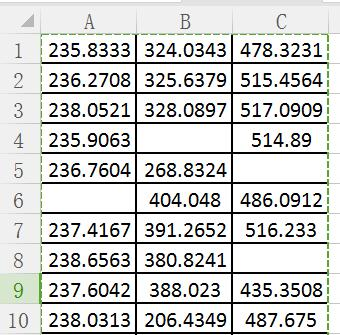具体代码如下所示：

[python]
view plain
copy

print
?

#读取数据 header设置Excel无标题头  import pandas as pd  data = pd.read_excel(”missing_data.xls”, header=None)   print data    #计算数据长度  print u‘行数’, len(data)    #计算用户A\B\C用电总和  print data.sum()    #计算用户A\B\C用点量算术平均数  mm = data.sum()  print mm    #输出预览前5行数据  print u‘预览前5行数据’  print data.head()    #输出数据基本统计量  print u‘输出数据基本统计量’  print data.describe()

#读取数据 header设置Excel无标题头
import pandas as pd
print data

 #计算数据长度 print u'行数', len(data) #计算用户A\B\C用电总和 print data.sum() #计算用户A\B\C用点量算术平均数 mm = data.sum() print mm #输出预览前5行数据 print u'预览前5行数据' print data.head() #输出数据基本统计量 print u'输出数据基本统计量' print data.describe()
输出结果如下所示：

[python]
view plain
copy

print
?

0         1         2  0   235.8333  324.0343  478.3231  1   236.2708  325.6379  515.4564  2   238.0521  328.0897  517.0909  3   235.9063       NaN  514.8900  4   236.7604  268.8324       NaN  5        NaN  404.0480  486.0912  6   237.4167  391.2652  516.2330  7   238.6563  380.8241       NaN  8   237.6042  388.0230  435.3508  …  行数 21  0    4488.9899  1    6182.3265  2    9416.3276  dtype: float64  0    4488.9899  1    6182.3265  2    9416.3276  dtype: float64  预览前5行数据            0         1         2  0  235.8333  324.0343  478.3231  1  236.2708  325.6379  515.4564  2  238.0521  328.0897  517.0909  3  235.9063       NaN  514.8900  4  236.7604  268.8324       NaN  输出数据基本统计量                  0           1           2  count   19.000000   17.000000   17.000000  mean   236.262626  363.666265  553.901624  std      1.225465   57.600529   67.707729  min    234.468800  206.434900  435.350800  25%           NaN         NaN         NaN  50%           NaN         NaN         NaN  75%           NaN         NaN         NaN  max    238.656300  416.879500  660.234700

           0         1         2
0   235.8333  324.0343  478.3231
1   236.2708  325.6379  515.4564
2   238.0521  328.0897  517.0909
3   235.9063       NaN  514.8900
4   236.7604  268.8324       NaN
5        NaN  404.0480  486.0912
6   237.4167  391.2652  516.2330
7   238.6563  380.8241       NaN
8   237.6042  388.0230  435.3508
...
行数 21
0    4488.9899
1    6182.3265
2    9416.3276
dtype: float64
0    4488.9899
1    6182.3265
2    9416.3276
dtype: float64
预览前5行数据
0         1         2
0  235.8333  324.0343  478.3231
1  236.2708  325.6379  515.4564
2  238.0521  328.0897  517.0909
3  235.9063       NaN  514.8900
4  236.7604  268.8324       NaN
输出数据基本统计量
0           1           2
count   19.000000   17.000000   17.000000
mean   236.262626  363.666265  553.901624
std      1.225465   57.600529   67.707729
min    234.468800  206.434900  435.350800
25%           NaN         NaN         NaN
50%           NaN         NaN         NaN
75%           NaN         NaN         NaN
max    238.656300  416.879500  660.234700
其中data.describe()输出数据的基本信息统计，其方法参考前面的图，包括count计数、std、max等函数。同时因为Excel表格中存在空值，故Python显示为NaN（Not a Number）表示空。
2.Series        Series是一维标记数组，可以存储任意数据类型，如整型、字符串、浮点型和Python对象等，轴标一般指索引。        Series、Numpy中的一维array 、Python基本数据结构List区别：List中的元素可以是不同的数据类型，而Array和Series中则只允许存储相同的数据类型，这样可以更有效的使用内存，提高运算效率。

[python]
view plain
copy

print
?

from pandas import Series, DataFrame    #通过传递一个list对象来创建Series，默认创建整型索引；  a = Series([4, 7, -5, 3])  print u‘创建Series:’  print a    #创建一个带有索引来确定每一个数据点的Series ;  b = Series([4, 7, -5, 3], index=[‘d’, ‘b’, ‘a’, ‘c’])  print u‘创建带有索引的Series:’  print b    #如果你有一些数据在一个Python字典中，你可以通过传递字典来创建一个Series；  sdata = {’Ohio’: 35000, ‘Texas’: 71000, ‘Oregon’: 16000, ‘Utah’: 5000}  c = Series(sdata)  print u‘通过传递字典创建Series:’  print c  states = [’California’, ‘Ohio’, ‘Oregon’, ‘Texas’]  d = Series(sdata, index=states)  print u‘California没有字典为空:’  print d

from pandas import Series, DataFrame

 #通过传递一个list对象来创建Series，默认创建整型索引； a = Series([4, 7, -5, 3]) print u'创建Series:' print a #创建一个带有索引来确定每一个数据点的Series ; b = Series([4, 7, -5, 3], index=['d', 'b', 'a', 'c']) print u'创建带有索引的Series:' print b #如果你有一些数据在一个Python字典中，你可以通过传递字典来创建一个Series； sdata = {'Ohio': 35000, 'Texas': 71000, 'Oregon': 16000, 'Utah': 5000} c = Series(sdata) print u'通过传递字典创建Series:' print c states = ['California', 'Ohio', 'Oregon', 'Texas'] d = Series(sdata, index=states) print u'California没有字典为空:' print d
输出如下所示：

[python]
view plain
copy

print
?

创建Series:  0    4  1    7  2   -5  3    3  dtype: int64  创建带有索引的Series:  d    4  b    7  a   -5  c    3  dtype: int64  通过传递字典创建Series:  Ohio      35000  Oregon    16000  Texas     71000  Utah       5000  dtype: int64  California没有字典为空:  California        NaN  Ohio          35000.0  Oregon        16000.0  Texas         71000.0  dtype: float64

创建Series:
0    4
1    7
2   -5
3    3
dtype: int64
创建带有索引的Series:
d    4
b    7
a   -5
c    3
dtype: int64
通过传递字典创建Series:
Ohio      35000
Oregon    16000
Texas     71000
Utah       5000
dtype: int64
California没有字典为空:
California        NaN
Ohio          35000.0
Oregon        16000.0
Texas         71000.0
dtype: float64
Series的一个重要功能是在算术运算中它会自动对齐不同索引的数据。

3.DataFrame              DataFrame是二维标记数据结构，列可以是不同的数据类型。它是最常用的pandas对象，像Series一样可以接收多种输入：lists、dicts、series和DataFrame等。初始化对象时，除了数据还可以传index和columns这两个参数。        注意：        (1) 在pandas中用函数 isnull 和 notnull 来检测数据丢失：pd.isnull(a)、pd.notnull(b)。        Series也提供了这些函数的实例方法：a.isnull()。        (2) Pandas提供了大量的方法能够轻松的对Series，DataFrame和Panel对象进行各种符合各种逻辑关系的合并操作。如：Concat、Merge （类似于SQL类型的合并）、Append （将一行连接到一个DataFrame上）。        (3) DataFrame中常常会出现重复行，DataFrame的duplicated方法返回一个布尔型Series，表示各行是否是重复行；还有一个drop_duplicated方法，它返回一个移除了重复行的DataFrame。        总之，Pandas是非常强大的一个数据分析包，很多功能都需要我自己去慢慢摸索。

四. Matplotlib画图包
Matplotlib是一个Python的图形框架，类似于MATLAB和R语言。它是python最著名的绘图库，它提供了一整套和matlab相似的命令API，十分适合交互式地进行制图。而且也可以方便地将它作为绘图控件，嵌入GUI应用程序中。        补充两张图，原自《Python数据分析与挖掘实战》，对大家绘图很有帮助。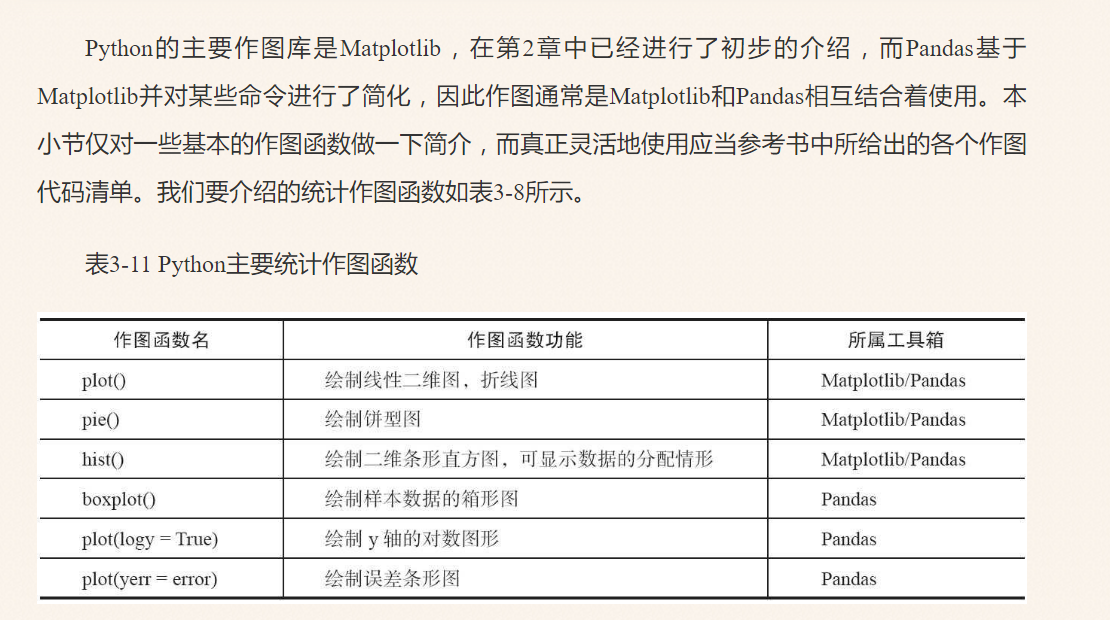最常用的画图函数是plot，同时常用的设置样式方法见下图。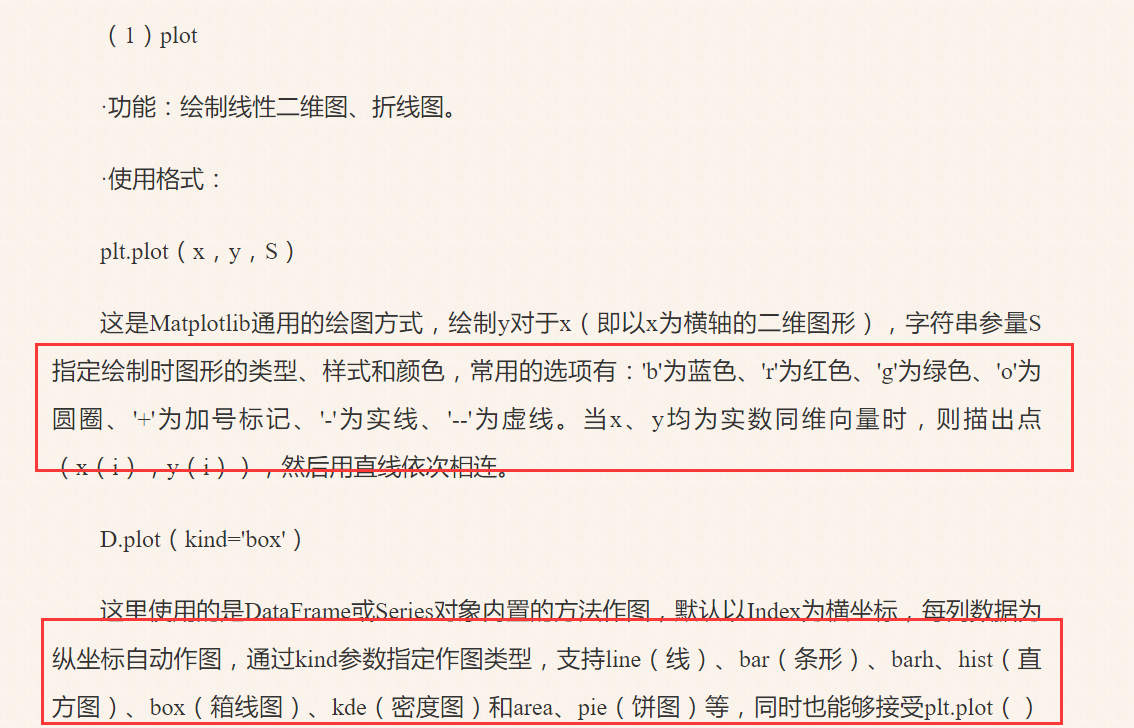这里主要使用前面第三部分Pandas读取的电力数据绘制图形，主要是柱状图和饼图。

1.绘制柱状图

[python]
view plain
copy

print
?

# -*- coding: utf-8 -*-  ”“” Created on Mon Nov 14 04:06:01 2016  @author: yxz15 ”“”    #导入数据集  import pandas as pd  data = pd.read_excel(”missing_data.xls”, header=None)   mm = data.sum()  print u‘计算用电量总数:’  print mm    #绘制图形  import numpy as np  import matplotlib.pyplot as plt  #中文字体显示  plt.rc(’font’, family=‘SimHei’, size=13)  N = 3  #3个用户 0 1 2  ind = np.arange(N)  # the x locations for the groups   print ind  #设置宽度  width = 0.35          x = [u’用户A’, u‘用户B’, u‘用户C’]  #绘图  plt.bar(ind, mm, width, color=’r’, label=‘sum num’)  plt.xlabel(u”用户名”)  plt.ylabel(u”总耗电量”)  plt.title(u’电力窃漏电用户自动识别–总耗电量’)  plt.legend()  #设置底部名称  plt.xticks(ind+width/2, x, rotation=40) #旋转40度  plt.show()

# -*- coding: utf-8 -*-
"""
Created on Mon Nov 14 04:06:01 2016

@author: yxz15
"""

 #导入数据集 import pandas as pd data = pd.read_excel("missing_data.xls", header=None) mm = data.sum() print u'计算用电量总数:' print mm #绘制图形 import numpy as np import matplotlib.pyplot as plt #中文字体显示 plt.rc('font', family='SimHei', size=13) N = 3 #3个用户 0 1 2 ind = np.arange(N) # the x locations for the groups print ind #设置宽度 width = 0.35 x = [u'用户A', u'用户B', u'用户C'] #绘图 plt.bar(ind, mm, width, color='r', label='sum num') plt.xlabel(u"用户名") plt.ylabel(u"总耗电量") plt.title(u'电力窃漏电用户自动识别--总耗电量') plt.legend() #设置底部名称 plt.xticks(ind+width/2, x, rotation=40) #旋转40度 plt.show()
输出如下所示：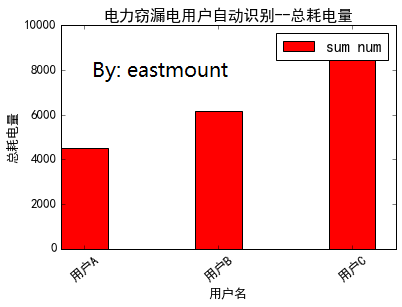2.绘制饼图

[python]
view plain
copy

print
?

import matplotlib.pyplot as plt    fracs = [45, 30, 25]             #每一块占得比例，总和为100  n = mm+mm+mm  a = (mm*1.0*100/n)  b = (mm*1.0*100/n)  c = (mm*1.0*100/n)  print a, b, c, n  fracs = [a, b, c]    explode=(0, 0, 0.08)             #离开整体的距离，看效果  labels = ’A’, ‘B’, ‘C’           #对应每一块的标志    plt.pie(fracs, explode=explode, labels=labels,                  autopct=’%1.1f%%’, shadow=True, startangle=90, colors = (“g”, “r”, “y”))                                   # startangle是开始的角度，默认为0，从这里开始按逆时针方向依次展开    plt.title(’Raining Hogs and Dogs’)   #标题    plt.show()

import matplotlib.pyplot as plt

fracs = [45, 30, 25]             #每一块占得比例，总和为100
n = mm+mm+mm
a = (mm*1.0*100/n)
b = (mm*1.0*100/n)
c = (mm*1.0*100/n)
print a, b, c, n
fracs = [a, b, c]

explode=(0, 0, 0.08)             #离开整体的距离，看效果
labels = 'A', 'B', 'C'           #对应每一块的标志

plt.pie(fracs, explode=explode, labels=labels,
autopct='%1.1f%%', shadow=True, startangle=90, colors = ("g", "r", "y"))
# startangle是开始的角度，默认为0，从这里开始按逆时针方向依次展开

plt.title('Raining Hogs and Dogs')   #标题

plt.show()
输出如下所示：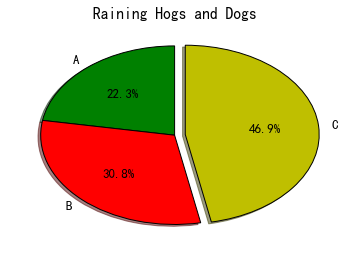3.柱状图及比例显示

[python]
view plain
copy

print
?

import matplotlib.pyplot as plt  import numpy as np  plt.rc(’font’, family=‘SimHei’, size=13)    num = np.array([13325, 9403, 9227, 8651])  ratio = np.array([0.75, 0.76, 0.72, 0.75])  men = num * ratio  women = num * (1-ratio)  x = [u’聊天’,u‘支付’,u‘团购\n优惠券’,u‘在线视频’]    width = 0.5  idx = np.arange(len(x))  plt.bar(idx, men, width, color=’red’, label=u‘男性用户’)  plt.bar(idx, women, width, bottom=men, color=’yellow’, label=u‘女性用户’)  plt.xlabel(u’应用类别’)  plt.ylabel(u’男女分布’)  plt.xticks(idx+width/2, x, rotation=40)  plt.legend()  plt.show()

import matplotlib.pyplot as plt
import numpy as np
plt.rc('font', family='SimHei', size=13)

num = np.array([13325, 9403, 9227, 8651])
ratio = np.array([0.75, 0.76, 0.72, 0.75])
men = num * ratio
women = num * (1-ratio)
x = [u'聊天',u'支付',u'团购\n优惠券',u'在线视频']

width = 0.5
idx = np.arange(len(x))
plt.bar(idx, men, width, color='red', label=u'男性用户')
plt.bar(idx, women, width, bottom=men, color='yellow', label=u'女性用户')
plt.xlabel(u'应用类别')
plt.ylabel(u'男女分布')
plt.xticks(idx+width/2, x, rotation=40)
plt.legend()
plt.show()
输出如下所示（PS：该部分参考百度知道，网址忘记了，望提醒）。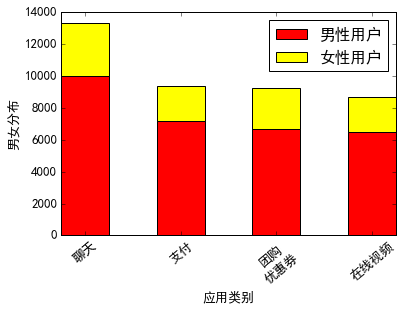当然该包可以绘制更多的图形，希望读者自己去学习。比如线性回归：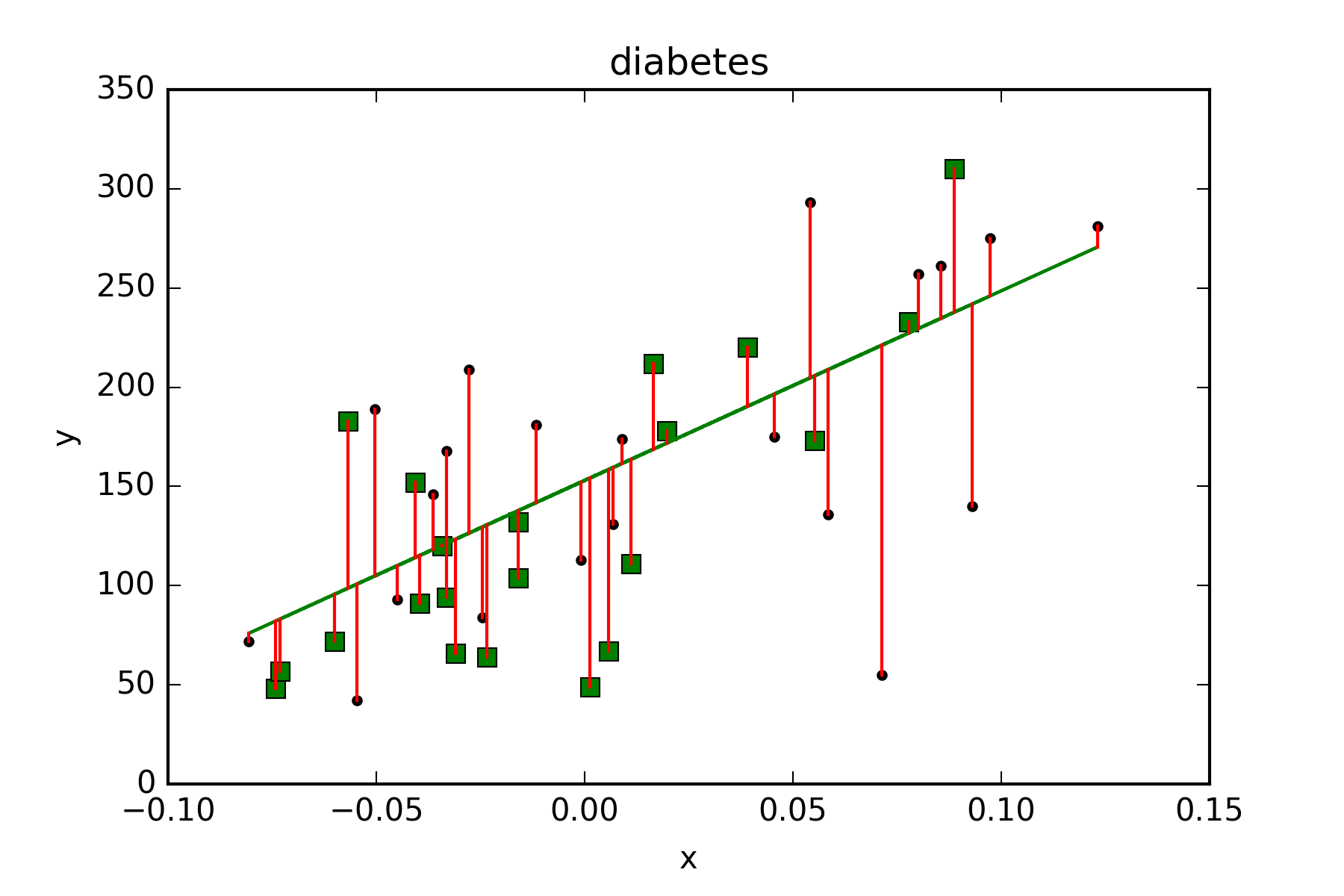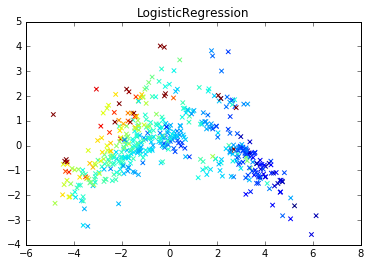代码部分详解，引用前面自己写的第三篇文章：        matplotlib.pyplot是用来画图的方法，matplotlib是可视化包。        import matplotlib.pyplot as plt
绘制散点图（scatter），横轴为x，获取的第1列数据；纵轴为y，获取的第2列数据；c=y_pred对聚类的预测结果画出散点图，marker=’o’说明用点表示图形。        plt.scatter(x, y, c=y_pred, marker=’o’)
表示图形x轴的标题。        plt.xlabel(“assists_per_minute”)
表示图形y轴的标题。        plt.ylabel(“points_per_minute”)
设置右上角图例。        plt.legend([“Rank”])
表示显示图形。        plt.show()

最后希望文章对你有所帮助，上课内容还需要继续探索，但enjoy myself~        同时周末监考两天回来，确实挺累的，事情堆了很多，浪费15个小时，发现这份工作，赚点外块真不容易啊！甚至比程序猿累多了。        当老师难，当好老师更难，当贵州的好老师难上难。希望还能坚持自己的梦想，做个财大信院的扫地僧吧，但每每和学生一起还是非常享受的。同时，这次熬夜写文到深夜4点半，旁边也坐着一个自己的学生，在调试Struts、Json代码，所以说，还真不是这边的学生差，你懂得，但也并不是没有好老师，只是相对较少。fighting~
最后补充学生冯Y的一首朋友圈感言：        把握现在，活在当下。        不以物喜，不以己悲。        闲看花开花落，        静观云卷云舒。        顺其自然，随遇而安。        我也希望自己有朝一日能达到这种心境~        对这份工作、事业、校园、办公还是得看淡点。

(By:Eastmount 2016-11-14 中午4点半
http://blog.csdn.net/eastmount/

)


展开全文python numpy
• 之后，美国国家航空航天局（NASA）太空望远镜科学研究所的哈勃望远镜背后团队（Hubble Space Telescope），选择Matplotlib作为了画图程序，并一直为 Matplotlib 开发团队提供资金支持，从而大大促进了 Matplotlib...数据可视化 python 数据分析
• 0. 安装并导入 安装： 打开终端输入pip3 install matplotlib 导入： import matlpotlib as plt 1. 绘图 首先无论绘制什么图最后都得加上一句： plt.show() 图像才能显示。
• 前面几篇文章采用的案例的方法进行介绍的，这篇文章主要介绍Python常用的扩展，同时结合数据挖掘相关知识介绍该具体的用法，主要介绍Numpy、Pandas和Matplotlib三个。目录： 一.Python常用扩展 二.Numpy...python 数据挖掘 numpy pandas
• 前一篇文章讲述了数据预处理、Jieba分词和文本聚类知识，这篇文章主要介绍Matplotlib和Pandas扩展绘图的基础用法，同时引入Echarts技术，该技术主要应用于网站可视化展示中。本文内容以实例为主，给读者最直观的...可视化分析 Python Pandas Echarts
• python里面的matplotlib.pylot是大家比较常用的，功能也还不错的一个。基本框架比较简单，但是做一个功能完善且比较好看整洁的图，免不了要网上查找一些函数。于是，为了节省时间，可以一劳永逸。我把常用函数作了...
• 这些教程涵盖了使用 Matplotlib 创建可视化的基础知识，以及有效使用的一些最佳实践。 可以简单的绘制出直方图，功率图，条形图，误差图，散点图等。 ...
• pip install --user matplotlib 测试I一下 有一段空白 没有报错 安装完成 安装web应用的request 以及web的框架 pip install --user virtualenv pip install Django           ...
• 基于此，为了彻底解决这些痛点，笔者下定决心通读，相比于市面上的很多学习材料，官方文档的内容极为详细而全面，更重要的是，其阐明了matplotlib包的设计架构，这些对于我们掌握并应用是非常有帮助的。在通读
• 我们导入matplotlib时，推荐使用： import matplotlib as mpl 因此，简洁起见，在后面我们将经常使用 mpl 代表 matplotlib。 还将用： figure ，表示matplotlib绘制的图形； Image，表示位图图像，或栅格图像，...
• 一、Matplotlib简介与安装 　Matplotlib也就是Matrix Plot Library，顾名思义，是Python的绘图库。它可与NumPy一起使用，提供了一种有效的MATLAB开源替代方案。它也可以和图形工具一起使用，如PyQt和wxPython。 　...
• Python 3.7 Manuals（64-bit） Python 3.7 开发手册 Python 3.7（64-bit） 控制台中运行Python 二：更新pip和setuptools，安装NumPy，安装Matplotlib包 以下三个命令都是在控制台（windows中的cmd）中运行 更新...
• 安装python python安装教程 ...安装各种科学 参考博文： python安装numpy教程等其他...window环境下python安装numpy、scipy、matplotlib等科学计算 安装简述： 打开地址：https://www.lfd.uci.edu/~gohlke/python...
• 1、matplotlibmatplotlib是numpy的扩展，可以实现python框架下的可视化，类似MATLAB的图像可视化。2、基本操作2.1绘画直方图#matplotlib使用 import matplotlib.pyplot as plt from numpy.random import normal,...python 可视化
• 测验1：Python基本语法元素 知识点概要： 普遍认为Python语言诞生于1991年 Python语言中的缩进在程序中长度统一且强制使用，只要统一即可，不一定是4个空格（尽管这是惯例） IPO模型指：Input Process Output ...
•  在利用Matplotlib包进行绘图的时候，大多数的数据都是来自于文件的，所以学会从文件中读取数据并展示出来就显得尤为重要了。我们将使用两个方法分别对文件中的数据进行加载并通过图形将数据展示出来。  1. ...机器学习 Python
• matplotlib相关性Matplotlib is a widely-used Python data visualization library that provides a numerous selection of 2D and 3D plots that are very useful for data analysis and machine learning tasks....python
• 今天，我们就来了解一下python强大的绘图库——Matplotlib及Seaborn的使用。首先我们先来了解一下这两个强大的库。Matplotlib 支持 Python 语言的开源绘图库（可以说是python里最优秀的绘图库了），因为其...
• 由于matplotlib绘图的功能非常强大，如果可以嵌入到别的应用程序框架里，这时开发各种各样的应用程序就非常方便了，让应用程序绘图的功能更快速地实现，满足客户的要求。matplotlib支持以下框架： Embedding in GTK...可视化 tkinter python
• 导入matplotlib import matplotlib.pyplot as plt import matplotlib Figures对象包含一个或多个Asex对象 方法： matplotlib.rc(‘figure’, figsize = (14, 7)) 全局设置大小 matplotlib.rc(‘font’, size = ...
• 本计划接着 Figure, Axes 对象，讨论 Axis 对象和 Ticks 对象。因为涉及到坐标变换，因此先把 ...与其它绘图一样，Matplotlib 包含用于确定画布上绘制的所有元素的最终位置的任意几何变换的框架，以便在不同的坐python 可视化
• 尽管matplotlib提供了出色的通用绘图功能并支持许多GUI和非GUI后端，但它与任何特定的GUI工具都没有非常紧密的集成。 有了大量的绘图组件和选项，程序员不容易为每个状态选择绘图选项，并且最终用户也不容易操纵...
• 唯一需要“安装”的是，这个需要添加到 .bashrc 中的ROS_PACKAGE_PATH安装脚本会自动为您执行此操作，只需运行 ./install 卸载 要卸载它，请删除添加到 .bashrc 的行并删除此 repo。 跑步 要运行，请运行脚本 ...Python
• 写在前面图种类&图要素框架部分示例代码开放性探讨问题参考文档-------...使用R的同学，一定对ggplot爱不释手，python也有ggplot，笔者暂时没有研究，后期会推出python可视化系列之seaborn,ggplot以及一些交互......